# Lecture Power Point Chemistry The Molecular Nature of

• Slides: 87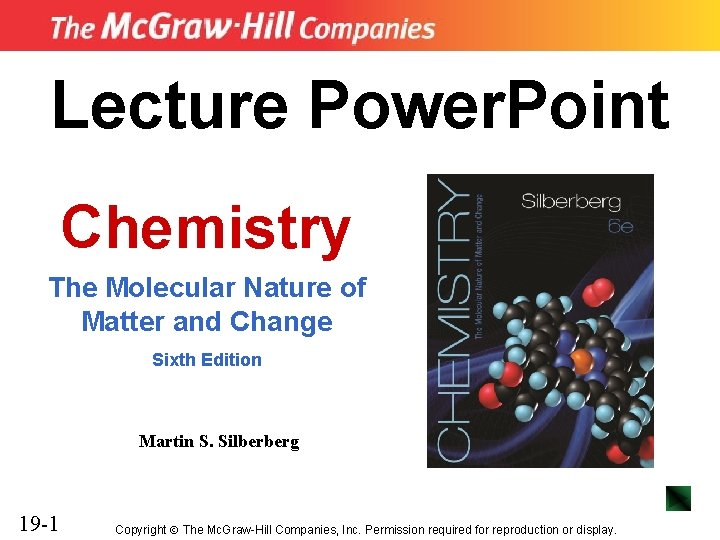Lecture Power. Point Chemistry The Molecular Nature of Matter and Change Sixth Edition Martin S. Silberberg 19 -1 Copyright The Mc. Graw-Hill Companies, Inc. Permission required for reproduction or display.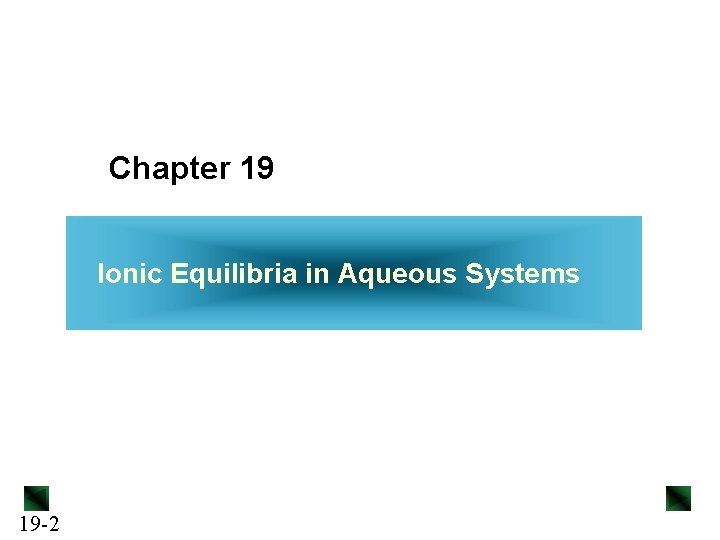Chapter 19 Ionic Equilibria in Aqueous Systems 19 -2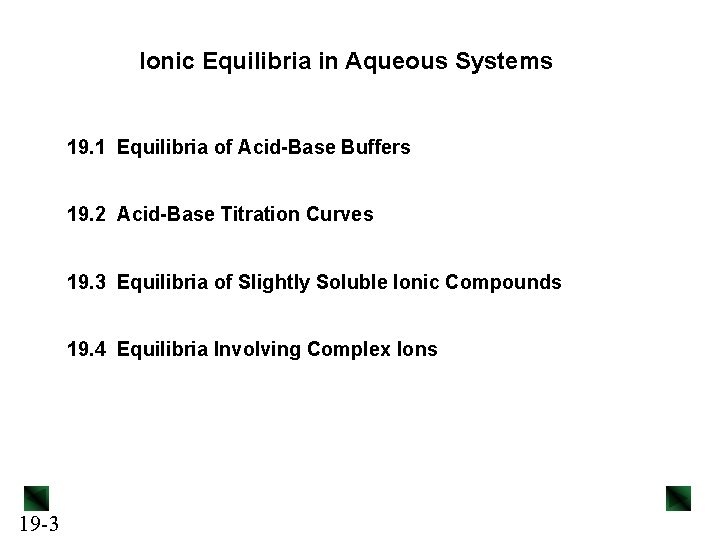Ionic Equilibria in Aqueous Systems 19. 1 Equilibria of Acid-Base Buffers 19. 2 Acid-Base Titration Curves 19. 3 Equilibria of Slightly Soluble Ionic Compounds 19. 4 Equilibria Involving Complex Ions 19 -3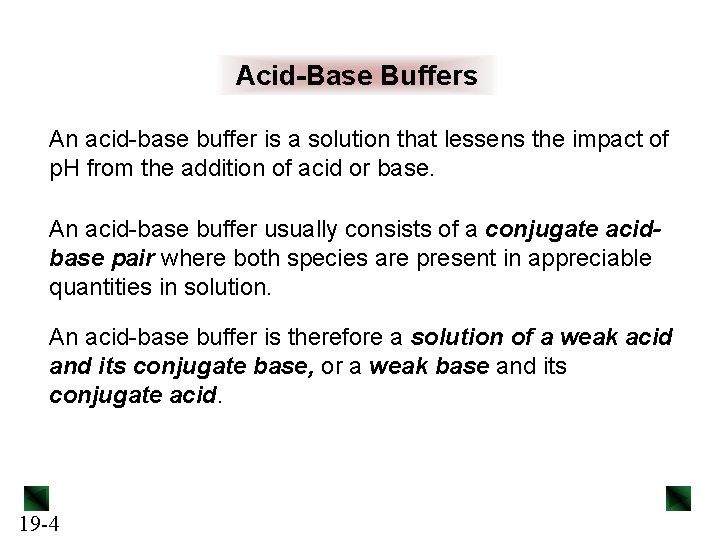Acid-Base Buffers An acid-base buffer is a solution that lessens the impact of p. H from the addition of acid or base. An acid-base buffer usually consists of a conjugate acidbase pair where both species are present in appreciable quantities in solution. An acid-base buffer is therefore a solution of a weak acid and its conjugate base, or a weak base and its conjugate acid. 19 -4Figure 19. 1 The effect of adding acid or base to an unbuffered solution. A 100 -m. L sample of dilute HCl is adjusted to p. H 5. 00. 19 -5 The addition of 1 m. L of strong acid (left) or strong base (right) changes the p. H by several units.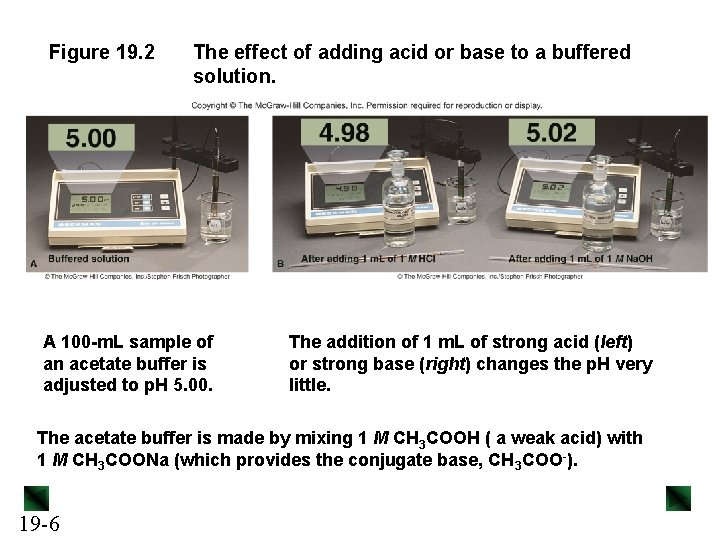Figure 19. 2 The effect of adding acid or base to a buffered solution. A 100 -m. L sample of an acetate buffer is adjusted to p. H 5. 00. The addition of 1 m. L of strong acid (left) or strong base (right) changes the p. H very little. The acetate buffer is made by mixing 1 M CH 3 COOH ( a weak acid) with 1 M CH 3 COONa (which provides the conjugate base, CH 3 COO-). 19 -6Buffers and the Common-ion Effect A buffer works through the common-ion effect. Acetic acid in water dissociates slightly to produce some acetate ion: CH 3 COOH(aq) + H 2 O(l) acetic acid CH 3 COO-(aq) + H 3 O+(aq) acetate ion If Na. CH 3 COO is added, it provides a source of CH 3 COO- ion, and the equilibrium shifts to the left. CH 3 COO- is common to both solutions. The addition of CH 3 COO- reduces the % dissociation of the acid. 19 -7Table 19. 1 The Effect of Added Acetate Ion on the Dissociation of Acetic Acid [CH 3 COOH]init [CH 3 COO-]added % Dissociation* [H 3 O+] p. H 0. 10 0. 00 1. 3 x 10 -3 2. 89 0. 10 0. 050 0. 036 3. 6 x 10 -5 4. 44 0. 10 0. 018 1. 8 x 10 -5 4. 74 0. 10 0. 15 0. 012 1. 2 x 1015 4. 92 * % Dissociation = 19 -8 [CH 3 COOH]dissoc [CH 3 COOH]init x 100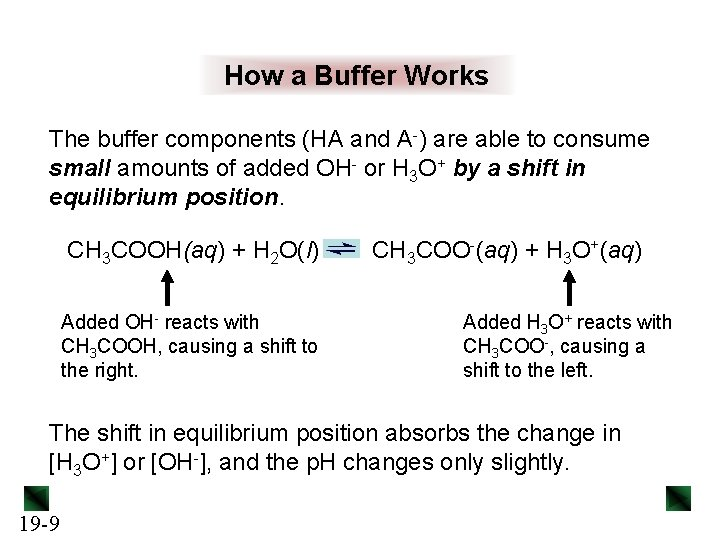How a Buffer Works The buffer components (HA and A-) are able to consume small amounts of added OH- or H 3 O+ by a shift in equilibrium position. CH 3 COOH(aq) + H 2 O(l) Added OH- reacts with CH 3 COOH, causing a shift to the right. CH 3 COO-(aq) + H 3 O+(aq) Added H 3 O+ reacts with CH 3 COO-, causing a shift to the left. The shift in equilibrium position absorbs the change in [H 3 O+] or [OH-], and the p. H changes only slightly. 19 -9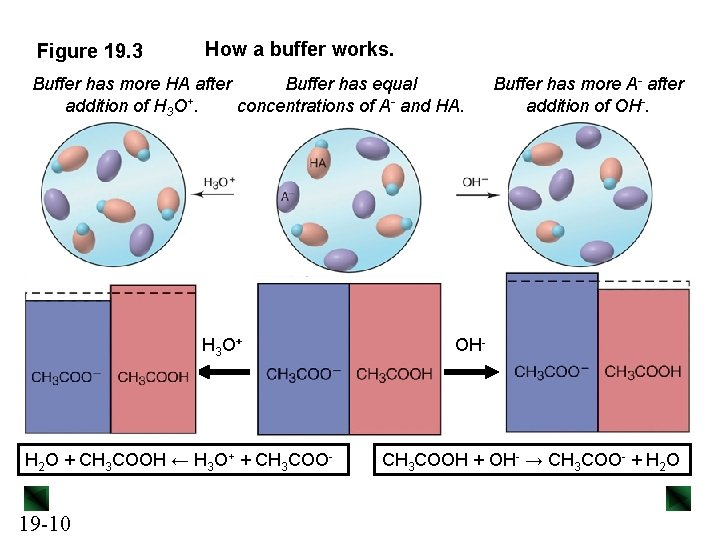Figure 19. 3 How a buffer works. Buffer has more HA after Buffer has equal addition of H 3 O+. concentrations of A- and HA. H 3 O + H 2 O + CH 3 COOH ← H 3 O+ + CH 3 COO- 19 -10 Buffer has more A- after addition of OH- CH 3 COOH + OH- → CH 3 COO- + H 2 ORelative Concentrations of Buffer Components CH 3 COOH(aq) + H 2 O(l) [CH 3 COO-][H 3 O+] Ka = [CH 3 COOH] CH 3 COO-(aq) + H 3 O+(aq) [H 3 O+] [CH 3 COOH] = Ka x [CH 3 COO-] Since Ka is constant, the [H 3 O+] of the solution depends on the ratio of buffer component concentrations. [HA] If the ratio - increases, [H 3 O+] increases. [A ] [HA] +] decreases. If the ratio decreases, [H O 3 [A-] 19 -11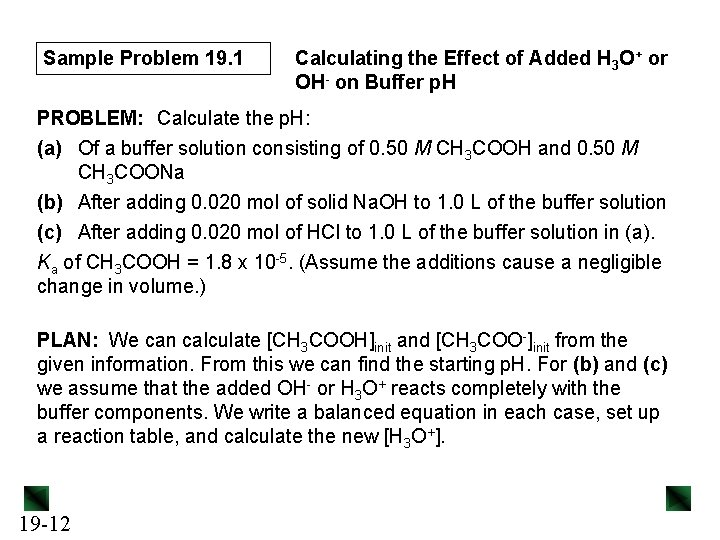Sample Problem 19. 1 Calculating the Effect of Added H 3 O+ or OH- on Buffer p. H PROBLEM: Calculate the p. H: (a) Of a buffer solution consisting of 0. 50 M CH 3 COOH and 0. 50 M CH 3 COONa (b) After adding 0. 020 mol of solid Na. OH to 1. 0 L of the buffer solution (c) After adding 0. 020 mol of HCl to 1. 0 L of the buffer solution in (a). Ka of CH 3 COOH = 1. 8 x 10 -5. (Assume the additions cause a negligible change in volume. ) PLAN: We can calculate [CH 3 COOH]init and [CH 3 COO-]init from the given information. From this we can find the starting p. H. For (b) and (c) we assume that the added OH- or H 3 O+ reacts completely with the buffer components. We write a balanced equation in each case, set up a reaction table, and calculate the new [H 3 O+]. 19 -12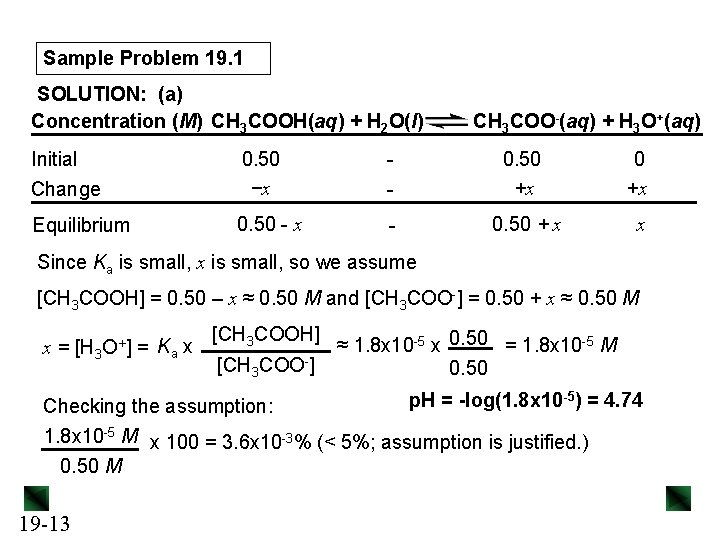Sample Problem 19. 1 SOLUTION: (a) Concentration (M) CH 3 COOH(aq) + H 2 O(l) Initial - Change 0. 50 −x Equilibrium 0. 50 - x CH 3 COO-(aq) + H 3 O+(aq) - 0. 50 +x - 0. 50 + x x Since Ka is small, x is small, so we assume [CH 3 COOH] = 0. 50 – x ≈ 0. 50 M and [CH 3 COO-] = 0. 50 + x ≈ 0. 50 M x = [H 3 O+] = Ka x [CH 3 COOH] [CH 3 COO-] ≈ 1. 8 x 10 -5 x 0. 50 = 1. 8 x 10 -5 M 0. 50 p. H = -log(1. 8 x 10 -5) = 4. 74 Checking the assumption: 1. 8 x 10 -5 M x 100 = 3. 6 x 10 -3% (< 5%; assumption is justified. ) 0. 50 M 19 -13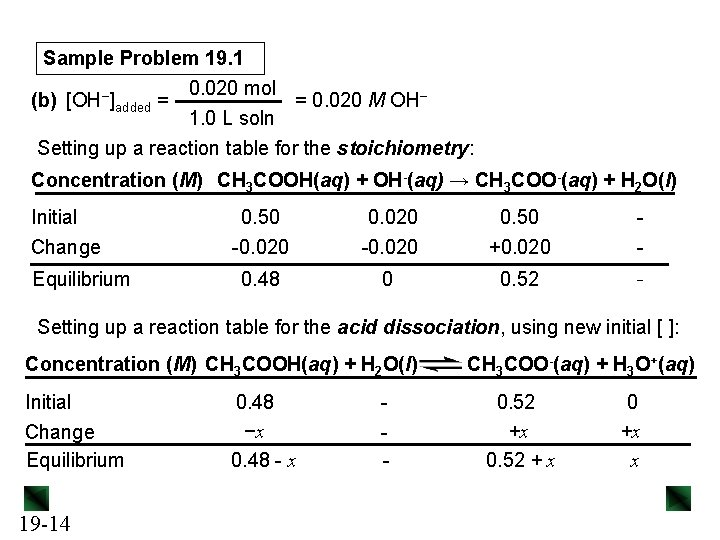Sample Problem 19. 1 0. 020 mol = 0. 020 M OH− (b) [OH−]added = 1. 0 L soln Setting up a reaction table for the stoichiometry: Concentration (M) CH 3 COOH(aq) + OH-(aq) → CH 3 COO-(aq) + H 2 O(l) Initial Change Equilibrium 0. 50 -0. 020 0. 50 +0. 020 - 0. 48 0 0. 52 - Setting up a reaction table for the acid dissociation, using new initial [ ]: Concentration (M) CH 3 COOH(aq) + H 2 O(l) Initial Change Equilibrium 19 -14 0. 48 −x 0. 48 - x - CH 3 COO-(aq) + H 3 O+(aq) 0. 52 +x 0. 52 + x 0 +x x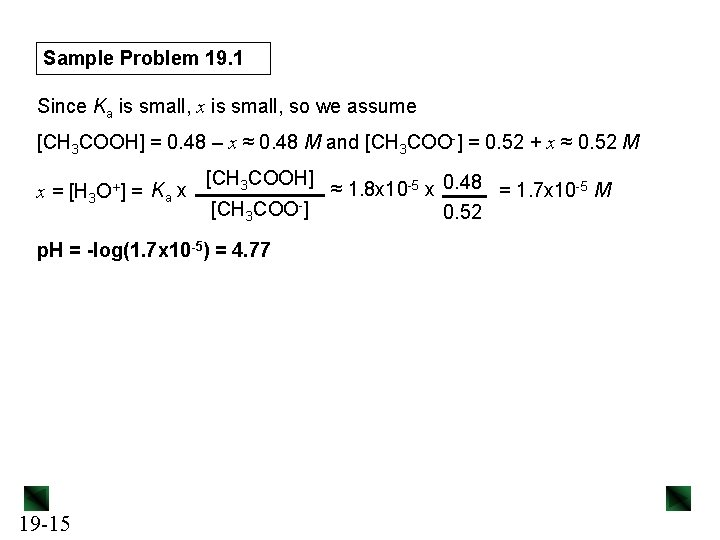Sample Problem 19. 1 Since Ka is small, x is small, so we assume [CH 3 COOH] = 0. 48 – x ≈ 0. 48 M and [CH 3 COO-] = 0. 52 + x ≈ 0. 52 M x = [H 3 O+] = Ka x [CH 3 COOH] [CH 3 COO-] p. H = -log(1. 7 x 10 -5) = 4. 77 19 -15 ≈ 1. 8 x 10 -5 x 0. 48 = 1. 7 x 10 -5 M 0. 52Sample Problem 19. 1 0. 020 mol = 0. 020 M H 3 O+ (c) [H 3 O+]added = 1. 0 L soln Setting up a reaction table for the stoichiometry: Concentration (M) CH 3 COO-(aq) + H 3 O+(aq) → CH 3 COOH(aq) + H 2 O(l) Initial Change Equilibrium 0. 50 -0. 020 0. 50 +0. 020 - 0. 48 0 0. 52 - Setting up a reaction table for the acid dissociation, using new initial [ ]: Concentration (M) CH 3 COOH(aq) + H 2 O(l) Initial Change Equilibrium 19 -16 0. 52 −x 0. 52 - x - CH 3 COO-(aq) + H 3 O+(aq) 0. 48 +x 0. 48 + x 0 +x xSample Problem 19. 1 Since Ka is small, x is small, so we assume [CH 3 COOH] = 0. 52 – x ≈ 0. 52 M and [CH 3 COO-] = 0. 48 + x ≈ 0. 48 M [CH 3 COOH] x = [H 3 O+] = Ka x ≈ 1. 8 x 10 -5 x 0. 52 = 2. 0 x 10 -5 M [CH 3 COO-] 0. 48 p. H = -log(2. 0 x 10 -5) = 4. 70 19 -17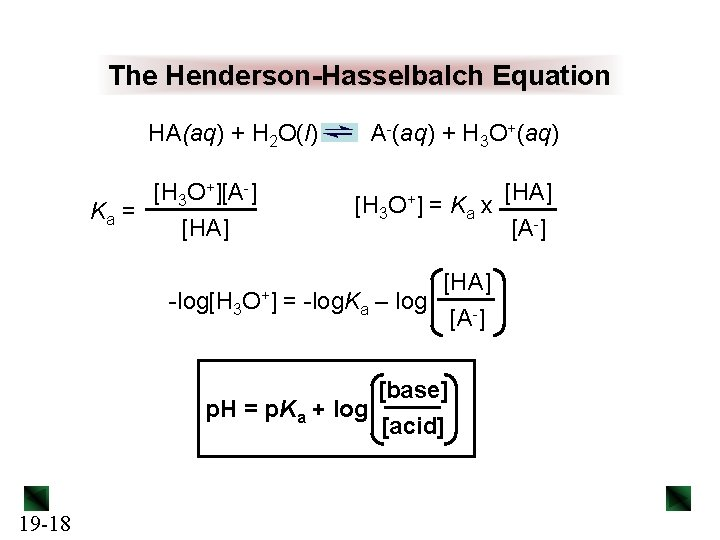The Henderson-Hasselbalch Equation HA(aq) + H 2 O(l) [H 3 O+][A-] Ka = [HA] -log[H 3 O+] A-(aq) + H 3 O+(aq) [H 3 O+] = Ka x [HA] = -log. Ka – log [A-] [base] p. H = p. Ka + log [acid] 19 -18 [HA] [A-]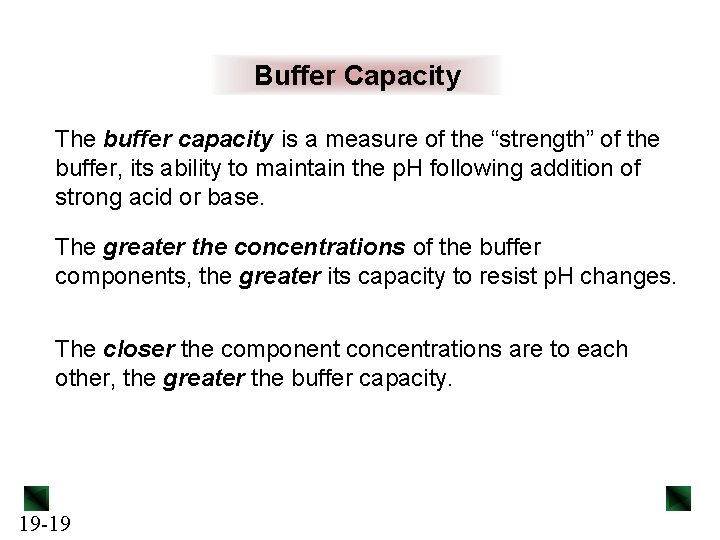Buffer Capacity The buffer capacity is a measure of the “strength” of the buffer, its ability to maintain the p. H following addition of strong acid or base. The greater the concentrations of the buffer components, the greater its capacity to resist p. H changes. The closer the component concentrations are to each other, the greater the buffer capacity. 19 -19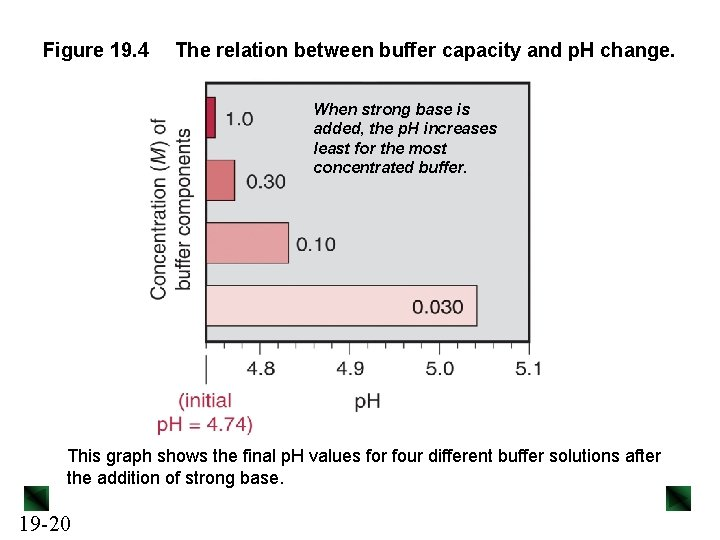Figure 19. 4 The relation between buffer capacity and p. H change. When strong base is added, the p. H increases least for the most concentrated buffer. This graph shows the final p. H values for four different buffer solutions after the addition of strong base. 19 -20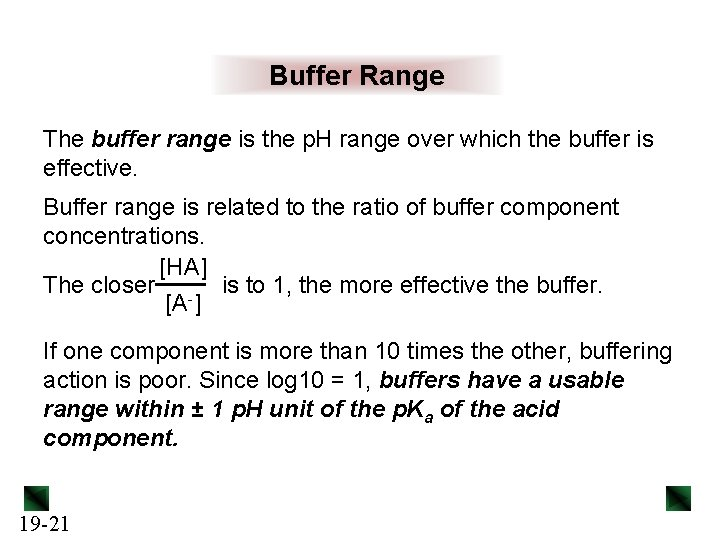Buffer Range The buffer range is the p. H range over which the buffer is effective. Buffer range is related to the ratio of buffer component concentrations. [HA] The closer - is to 1, the more effective the buffer. [A ] If one component is more than 10 times the other, buffering action is poor. Since log 10 = 1, buffers have a usable range within ± 1 p. H unit of the p. Ka of the acid component. 19 -21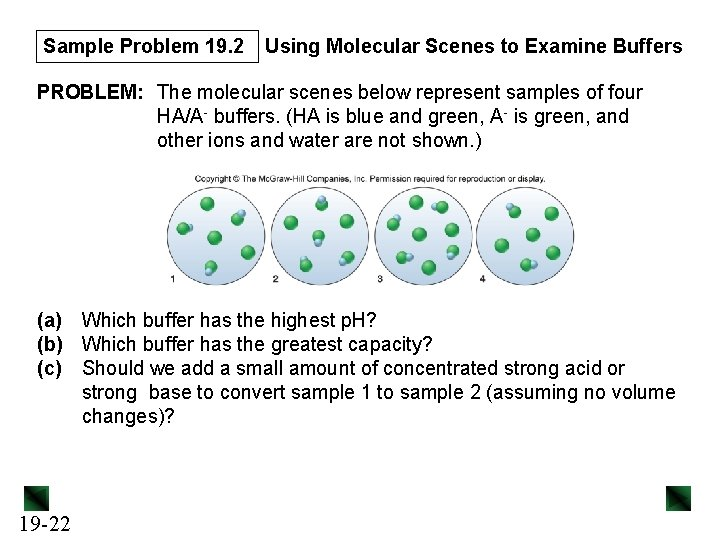Sample Problem 19. 2 Using Molecular Scenes to Examine Buffers PROBLEM: The molecular scenes below represent samples of four HA/A- buffers. (HA is blue and green, A- is green, and other ions and water are not shown. ) (a) Which buffer has the highest p. H? (b) Which buffer has the greatest capacity? (c) Should we add a small amount of concentrated strong acid or strong base to convert sample 1 to sample 2 (assuming no volume changes)? 19 -22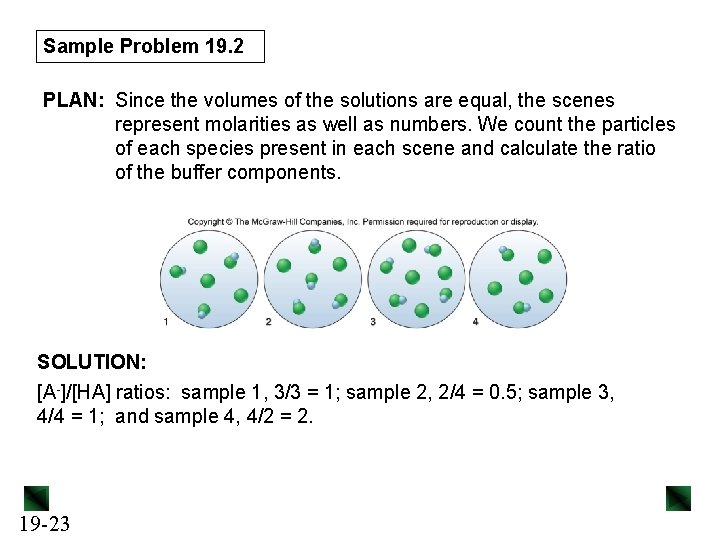Sample Problem 19. 2 PLAN: Since the volumes of the solutions are equal, the scenes represent molarities as well as numbers. We count the particles of each species present in each scene and calculate the ratio of the buffer components. SOLUTION: [A-]/[HA] ratios: sample 1, 3/3 = 1; sample 2, 2/4 = 0. 5; sample 3, 4/4 = 1; and sample 4, 4/2 = 2. 19 -23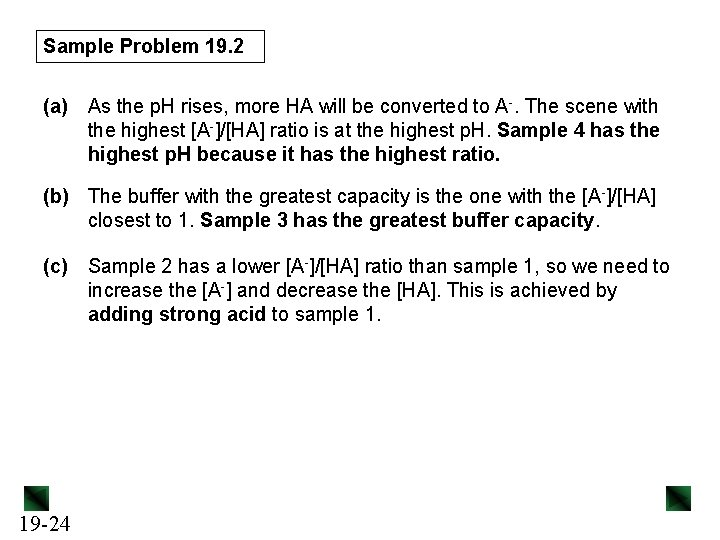Sample Problem 19. 2 (a) As the p. H rises, more HA will be converted to A-. The scene with the highest [A-]/[HA] ratio is at the highest p. H. Sample 4 has the highest p. H because it has the highest ratio. (b) The buffer with the greatest capacity is the one with the [A-]/[HA] closest to 1. Sample 3 has the greatest buffer capacity. (c) 19 -24 Sample 2 has a lower [A-]/[HA] ratio than sample 1, so we need to increase the [A-] and decrease the [HA]. This is achieved by adding strong acid to sample 1.Preparing a Buffer • Choose the conjugate acid-base pair. – The p. Ka of the weak acid component should be close to the desired p. H. • Calculate the ratio of buffer component concentrations. p. H = p. Ka + log [base] [acid] • Determine the buffer concentration, and calculate the required volume of stock solutions and/or masses of components. • Mix the solution and correct the p. H. 19 -25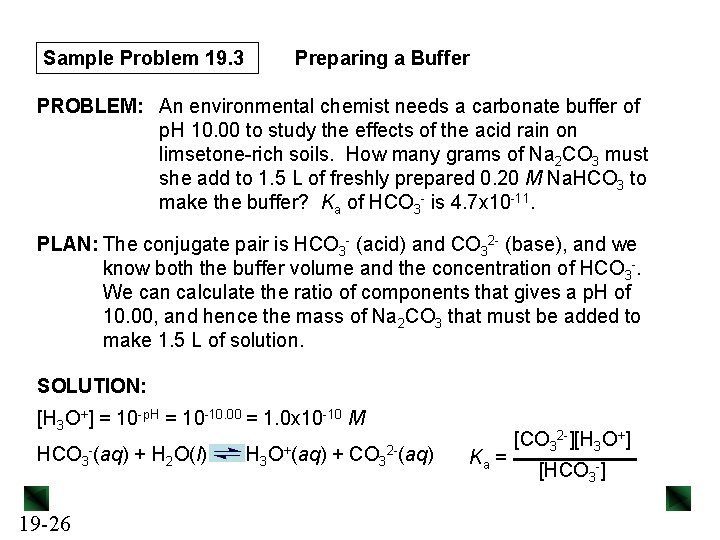Sample Problem 19. 3 Preparing a Buffer PROBLEM: An environmental chemist needs a carbonate buffer of p. H 10. 00 to study the effects of the acid rain on limsetone-rich soils. How many grams of Na 2 CO 3 must she add to 1. 5 L of freshly prepared 0. 20 M Na. HCO 3 to make the buffer? Ka of HCO 3 - is 4. 7 x 10 -11. PLAN: The conjugate pair is HCO 3 - (acid) and CO 32 - (base), and we know both the buffer volume and the concentration of HCO 3 -. We can calculate the ratio of components that gives a p. H of 10. 00, and hence the mass of Na 2 CO 3 that must be added to make 1. 5 L of solution. SOLUTION: [H 3 O+] = 10 -p. H = 10 -10. 00 = 1. 0 x 10 -10 M HCO 3 19 -26 -(aq) + H 2 O(l) H 3 O+(aq) + CO 3 2 -(aq) Ka = [CO 32 -][H 3 O+] [HCO 3 -]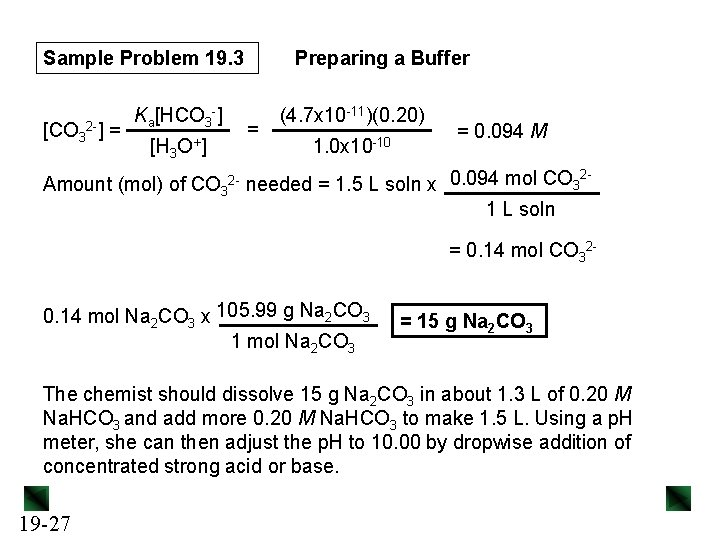Sample Problem 19. 3 [CO 3 2 -] = Ka[HCO 3 -] [H 3 O+ ] Preparing a Buffer = (4. 7 x 10 -11)(0. 20) 1. 0 x 10 -10 = 0. 094 M 2 Amount (mol) of CO 32 - needed = 1. 5 L soln x 0. 094 mol CO 3 1 L soln = 0. 14 mol CO 320. 14 mol Na 2 CO 3 x 105. 99 g Na 2 CO 3 1 mol Na 2 CO 3 = 15 g Na 2 CO 3 The chemist should dissolve 15 g Na 2 CO 3 in about 1. 3 L of 0. 20 M Na. HCO 3 and add more 0. 20 M Na. HCO 3 to make 1. 5 L. Using a p. H meter, she can then adjust the p. H to 10. 00 by dropwise addition of concentrated strong acid or base. 19 -27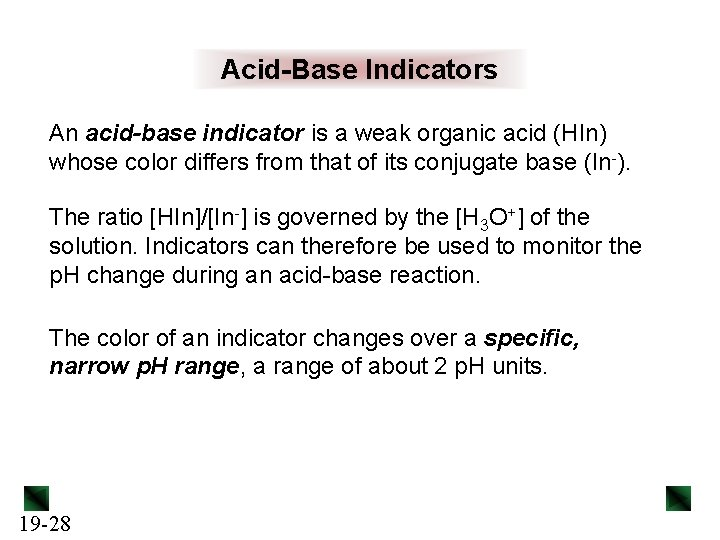Acid-Base Indicators An acid-base indicator is a weak organic acid (HIn) whose color differs from that of its conjugate base (In-). The ratio [HIn]/[In-] is governed by the [H 3 O+] of the solution. Indicators can therefore be used to monitor the p. H change during an acid-base reaction. The color of an indicator changes over a specific, narrow p. H range, a range of about 2 p. H units. 19 -28Figure 19. 5 Colors and approximate p. H range of some common acid-base indicators. p. H 19 -29Figure 19. 6 The color change of the indicator bromthymol blue. p. H < 6. 0 19 -30 p. H = 6. 0 -7. 5 p. H > 7. 5Acid-Base Titrations In an acid-base titration, the concentration of an acid (or a base) is determined by neutralizing the acid (or base) with a solution of base (or acid) of known concentration. The equivalence point of the reaction occurs when the number of moles of OH- added equals the number of moles of H 3 O+ originally present, or vice versa. The end point occurs when the indicator changes color. - The indicator should be selected so that its color change occurs at a p. H close to that of the equivalence point. 19 -31Figure 19. 7 Curve for a strong acid–strong base titration. The p. H increases gradually when excess base has been added. The p. H rises very rapidly at the equivalence point, which occurs at p. H = 7. 00. The initial p. H is low. 19 -32Calculating the p. H during a strong acid–strong base titration Initial p. H [H 3 O+] = [HA]init p. H = -log[H 3 O+] p. H before equivalence point initial mol H 3 O+ = Vacid x Macid mol OH- added = Vbase x Mbase mol H 3 O+remaining = (mol H 3 O+init) – (mol OH-added) [H 3 19 -33 O+ ] = mol H 3 O+remaining Vacid + Vbase p. H = -log[H 3 O+]Calculating the p. H during a strong acid–strong base titration p. H at the equivalence point p. H = 7. 00 for a strong acid-strong base titration. p. H beyond the equivalence point initial mol H 3 O+ = Vacid x Macid mol OH- added = Vbase x Mbase mol OH-excess = (mol OH-added) – (mol H 3 O+init) [OH-] = mol OH-excess Vacid + Vbase p. OH = -log[OH-] and p. H = 14. 00 - p. OH 19 -34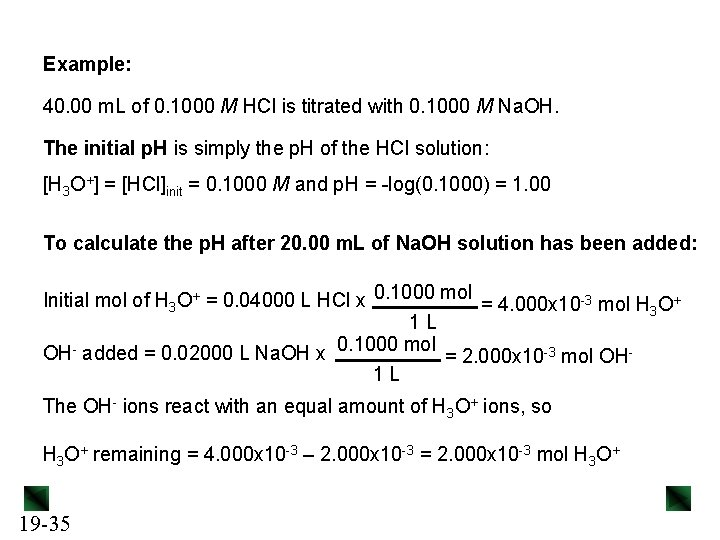Example: 40. 00 m. L of 0. 1000 M HCl is titrated with 0. 1000 M Na. OH. The initial p. H is simply the p. H of the HCl solution: [H 3 O+] = [HCl]init = 0. 1000 M and p. H = -log(0. 1000) = 1. 00 To calculate the p. H after 20. 00 m. L of Na. OH solution has been added: Initial mol of H 3 O+ = 0. 04000 L HCl x 0. 1000 mol = 4. 000 x 10 -3 mol H 3 O+ 1 L OH- added = 0. 02000 L Na. OH x 0. 1000 mol = 2. 000 x 10 -3 mol OH 1 L The OH- ions react with an equal amount of H 3 O+ ions, so H 3 O+ remaining = 4. 000 x 10 -3 – 2. 000 x 10 -3 = 2. 000 x 10 -3 mol H 3 O+ 19 -35[H 3 O+ ] 2. 000 x 10 -3 mol = = 0. 03333 M 0. 04000 L + 0. 02000 L p. H = -log(0. 03333) = 1. 48 The equivalence point occurs when mol of OH- added = initial mol of HCl, so when 40. 00 m. L of Na. OH has been added. To calculate the p. H after 50. 00 m. L of Na. OH solution has been added: OH- added = 0. 05000 L Na. OH x 0. 1000 mol = 5. 000 x 10 -3 mol OH 1 L OH- in excess = 5. 000 x 10 -3 – 4. 000 x 10 -3 = 1. 000 x 10 -3 mol OH[OH-] = 1. 000 x 10 -3 mol = 0. 01111 M 0. 04000 L + 0. 05000 L p. OH = -log(0. 01111) = 1. 95 19 -36 p. H = 14. 00 – 1. 95 = 12. 05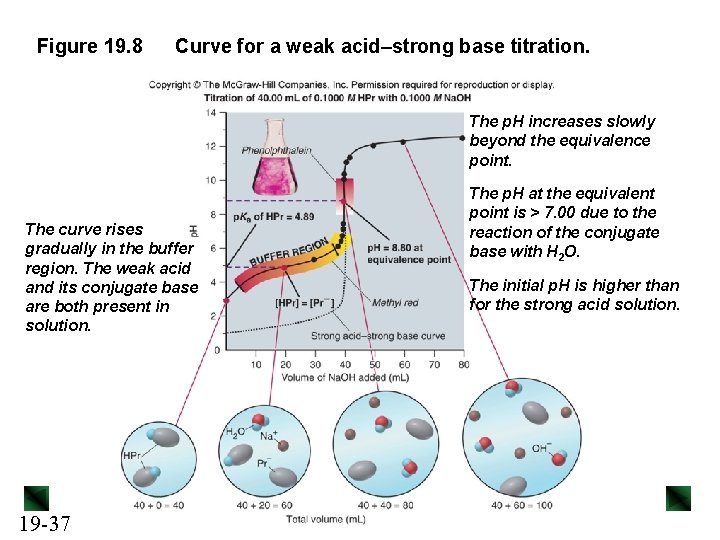Figure 19. 8 Curve for a weak acid–strong base titration. The p. H increases slowly beyond the equivalence point. The curve rises gradually in the buffer region. The weak acid and its conjugate base are both present in solution. 19 -37 The p. H at the equivalent point is > 7. 00 due to the reaction of the conjugate base with H 2 O. The initial p. H is higher than for the strong acid solution.Calculating the p. H during a weak acid–strong base titration Initial p. H Ka = [H 3 O+][A-] [HA] [H 3 O+] = p. H = -log[H 3 O+] p. H before equivalence point [H 3 O+] = Ka x [HA] [A-] p. H = p. Ka + log 19 -38 [base] [acid] or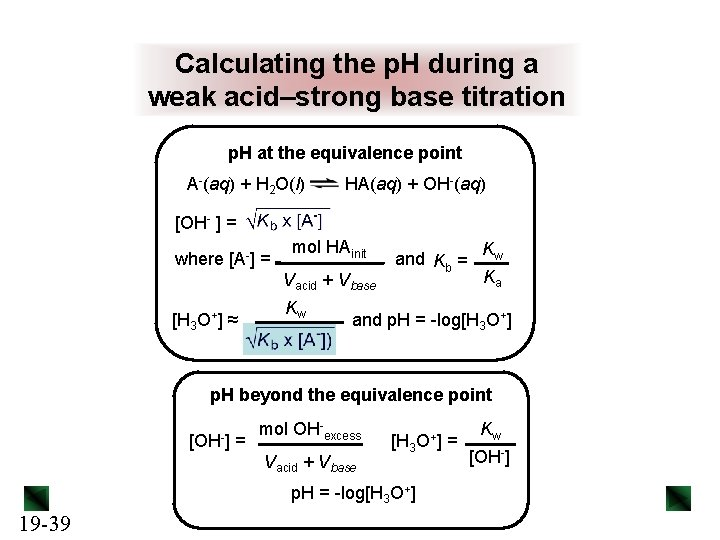Calculating the p. H during a weak acid–strong base titration p. H at the equivalence point A-(aq) + H 2 O(l) HA(aq) + OH-(aq) [OH- ] = where [A-] = mol HAinit Vacid + Vbase [H 3 O+] ≈ Kw and Kb = Kw Ka and p. H = -log[H 3 O+] p. H beyond the equivalence point [OH-] = mol OH-excess Vacid + Vbase [H 3 O+] = p. H = -log[H 3 O+] 19 -39 Kw [OH-]Sample Problem 19. 4 Finding the p. H During a Weak Acid– Strong Base Titration PROBLEM: Calculate the p. H during the titration of 40. 00 m. L of 0. 1000 M propanoic acid (HPr; Ka = 1. 3 x 10 -5) after adding the following volumes of 0. 1000 M Na. OH: (a) 0. 00 m. L (b) 30. 00 m. L (c) 40. 00 m. L (d) 50. 00 m. L PLAN: The initial p. H must be calculated using the Ka value for the weak acid. We then calculate the number of moles of HPr present initially and the number of moles of OH- added. Once we know the volume of base required to reach the equivalence point we can calculate the p. H based on the species present in solution. SOLUTION: (a) [H 3 O+] = p. H = -log(1. 1 x 10 -3) = 2. 96 19 -40 = 1. 1 x 10 -3 M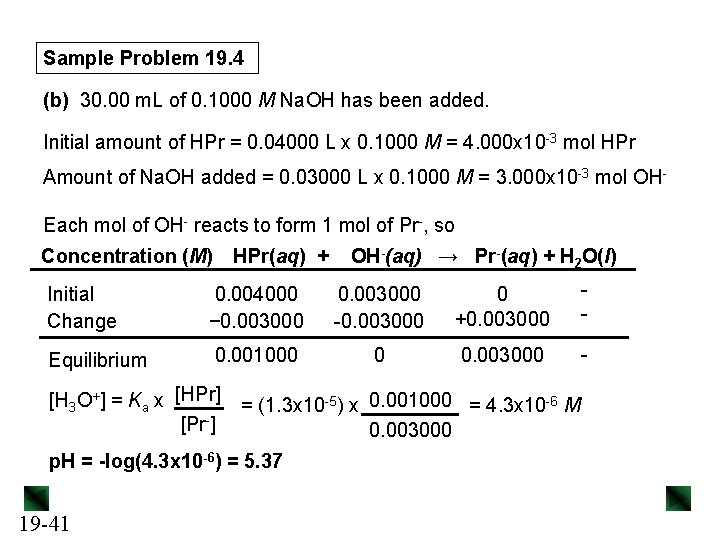Sample Problem 19. 4 (b) 30. 00 m. L of 0. 1000 M Na. OH has been added. Initial amount of HPr = 0. 04000 L x 0. 1000 M = 4. 000 x 10 -3 mol HPr Amount of Na. OH added = 0. 03000 L x 0. 1000 M = 3. 000 x 10 -3 mol OHEach mol of OH- reacts to form 1 mol of Pr-, so Concentration (M) HPr(aq) + OH-(aq) → Pr-(aq) + H 2 O(l) Initial Change 0. 004000 − 0. 003000 -0. 003000 0 +0. 003000 - Equilibrium 0. 001000 0 0. 003000 - [H 3 O+] = Ka x [HPr] [Pr-] = (1. 3 x 10 -5) x 0. 001000 = 4. 3 x 10 -6 M 0. 003000 p. H = -log(4. 3 x 10 -6) = 5. 37 19 -41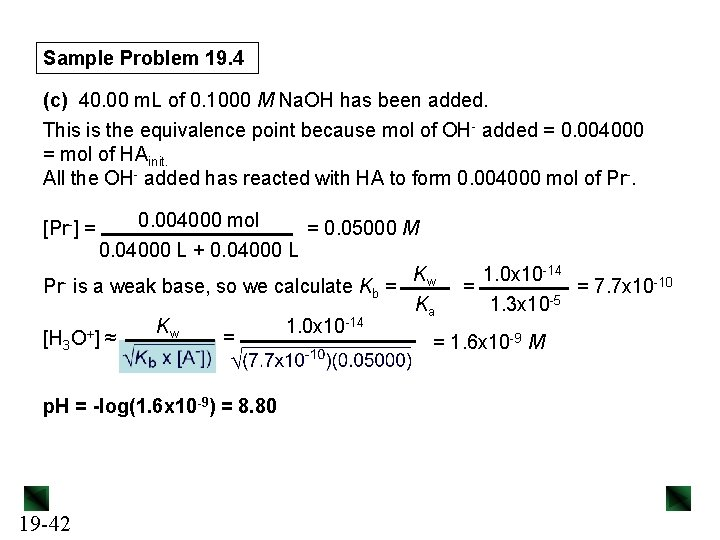Sample Problem 19. 4 (c) 40. 00 m. L of 0. 1000 M Na. OH has been added. This is the equivalence point because mol of OH- added = 0. 004000 = mol of HAinit. All the OH- added has reacted with HA to form 0. 004000 mol of Pr-. 0. 004000 mol = 0. 05000 M 0. 04000 L + 0. 04000 L Kw 1. 0 x 10 -14 Pr is a weak base, so we calculate Kb = = = 7. 7 x 10 -10 Ka 1. 3 x 10 -5 Kw 1. 0 x 10 -14 + = [H 3 O ] ≈ = 1. 6 x 10 -9 M [Pr-] = p. H = -log(1. 6 x 10 -9) = 8. 80 19 -42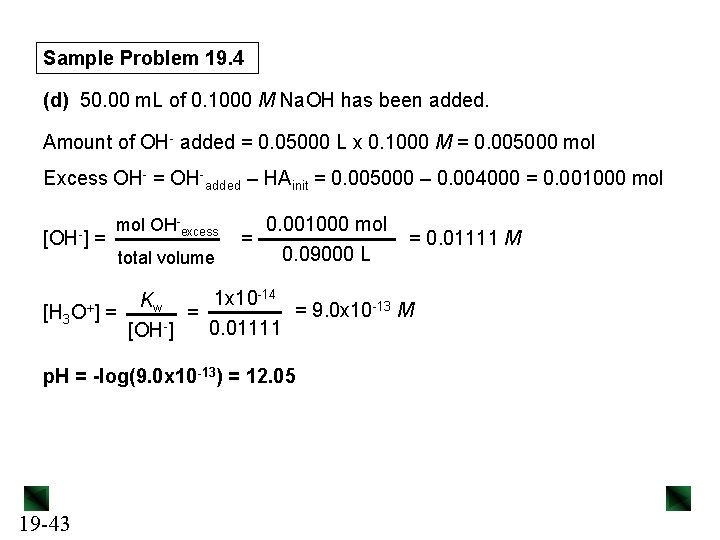Sample Problem 19. 4 (d) 50. 00 m. L of 0. 1000 M Na. OH has been added. Amount of OH- added = 0. 05000 L x 0. 1000 M = 0. 005000 mol Excess OH- = OH-added – HAinit = 0. 005000 – 0. 004000 = 0. 001000 mol [OH-] = mol OH-excess total volume 0. 001000 mol = 0. 09000 L = 0. 01111 M -14 1 x 10 K w = 9. 0 x 10 -13 M [H 3 O+] = = 0. 01111 [OH-] p. H = -log(9. 0 x 10 -13) = 12. 05 19 -43Figure 19. 9 Curve for a weak base–strong acid titration. The p. H decreases gradually in the buffer region. The weak base and its conjugate acid are both present in solution. 19 -44 The p. H at the equivalence point is < 7. 00 due to the reaction of the conjugate acid with H 2 O.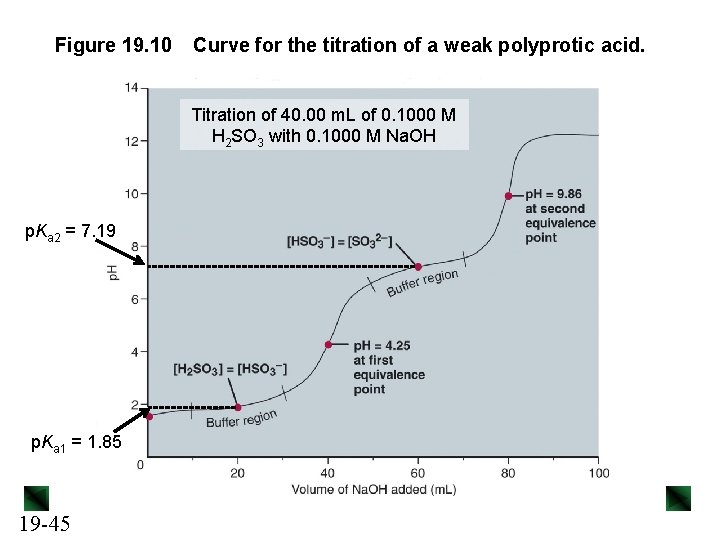Figure 19. 10 Curve for the titration of a weak polyprotic acid. Titration of 40. 00 m. L of 0. 1000 M H 2 SO 3 with 0. 1000 M Na. OH p. Ka 2 = 7. 19 p. Ka 1 = 1. 85 19 -45Amino Acids as Polyprotic Acids An amino acid contains a weak base (-NH 2) and a weak acid (-COOH) in the same molecule. Both groups are protonated at low p. H and the amino acid behaves like a polyprotic acid. 19 -46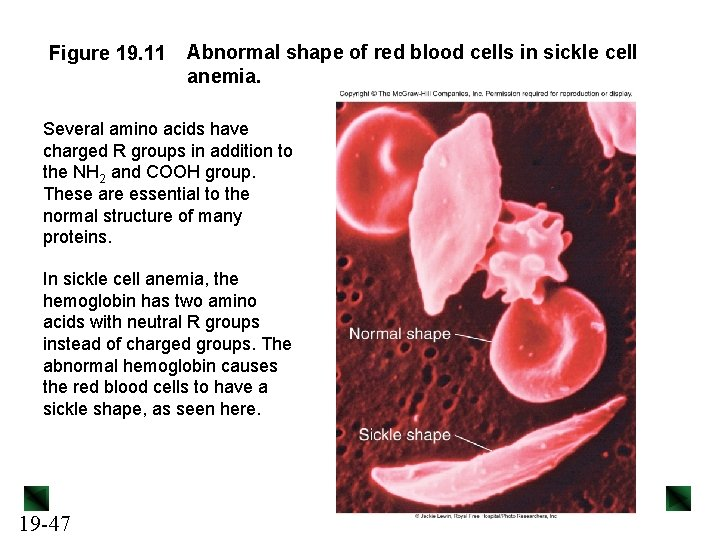Figure 19. 11 Abnormal shape of red blood cells in sickle cell anemia. Several amino acids have charged R groups in addition to the NH 2 and COOH group. These are essential to the normal structure of many proteins. In sickle cell anemia, the hemoglobin has two amino acids with neutral R groups instead of charged groups. The abnormal hemoglobin causes the red blood cells to have a sickle shape, as seen here. 19 -47Equilibria of Slightly Soluble Ionic Compounds Any “insoluble” ionic compound is actually slightly soluble in aqueous solution. We assume that the very small amount of such a compound that dissolves will dissociate completely. For a slightly soluble ionic compound in water, equilibrium exists between solid solute and aqueous ions. Pb. F 2(s) [Pb 2+][F-]2 Qc = [Pb. F 2] 19 -48 Pb 2+(aq) + 2 F-(aq) Qsp = Qc[Pb. F 2] = [Pb 2+][F-]2Qsp and Ksp Qsp is called the ion-product expression for a slightly soluble ionic compound. For any slightly soluble compound Mp. Xq, which consists of ions Mn+ and Xz-, Qsp = [Mn+]p[Xz-]q When the solution is saturated, the system is at equilibrium, and Qsp = Ksp, the solubility product constant. The Ksp value of a salt indicates how far the dissolution proceeds at equilibrium (saturation). 19 -49Metal Sulfides Metal sulfides behave differently from most other slightly soluble ionic compounds, since the S 2 - ion is strongly basic. We can think of the dissolution of a metal sulfide as a two -step process: Mn. S(s) Mn 2+(aq) + S 2 -(aq) + H 2 O(l) → HS-(aq) + OH-(aq) Mn. S(s) + H 2 O(l) Mn 2+(aq) + HS-(aq) + OH-(aq) Ksp = [Mn 2+][HS-][OH-] 19 -50Sample Problem 19. 5 Writing Ion-Product Expressions PROBLEM: Write the ion-product expression at equilibrium for each compound: (a) magnesium carbonate (b) iron(II) hydroxide (c) calcium phosphate (d) silver sulfide PLAN: We write an equation for a saturated solution of each compound, and then write the ion-product expression at equilibrium, Ksp. Note the sulfide in part (d). SOLUTION: (a) Mg. CO 3(s) (b) Fe(OH)2(s) (c) 19 -51 Ca 3(PO 4)2(s) Mg 2+(aq) + CO 32 -(aq) Fe 2+(aq) + 2 OH-(aq) 3 Ca 2+(aq) + 2 PO 43 -(aq) Ksp = [Mg 2+][CO 32 -] Ksp = [Fe 2+][OH-]2 Ksp = [Ca 2+]3[PO 43 -]2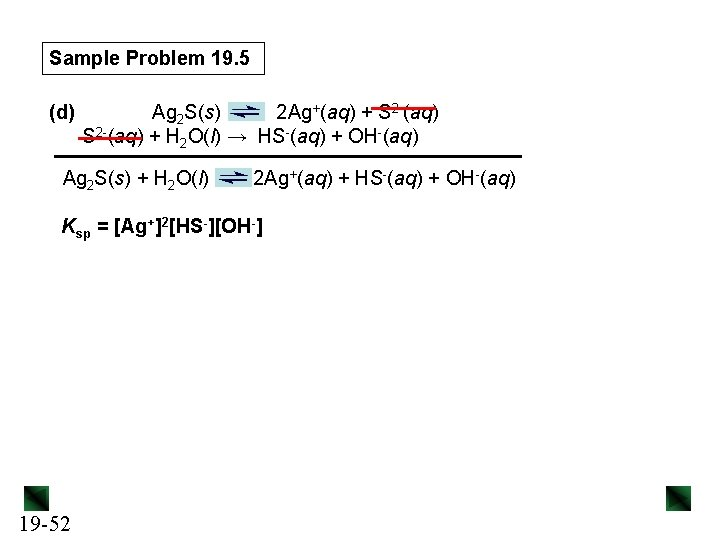Sample Problem 19. 5 (d) Ag 2 S(s) 2 Ag+(aq) + S 2 -(aq) + H 2 O(l) → HS-(aq) + OH-(aq) Ag 2 S(s) + H 2 O(l) 2 Ag+(aq) + HS-(aq) + OH-(aq) Ksp = [Ag+]2[HS-][OH-] 19 -52Table 19. 2 Solubility-Product Constants (Ksp) of Selected Ionic Compounds at 25°C Name, Formula 19 -53 Ksp Aluminum hydroxide, Al(OH)3 3 x 10 -34 Cobalt(II) carbonate, Co. CO 3 1. 0 x 10 -10 Iron(II) hydroxide, Fe(OH)2 4. 1 x 10 -15 Lead(II) fluoride, Pb. F 2 3. 6 x 10 -8 Lead(II) sulfate, Pb. SO 4 1. 6 x 10 -8 Mercury(I) iodide, Hg 2 I 2 4. 7 x 10 -29 Silver sulfide, Ag 2 S 8 x 10 -48 Zinc iodate, Zn(IO 3)2 3. 9 x 10 -6Sample Problem 19. 6 Determining Ksp from Solubility PROBLEM: (a) Lead(II) sulfate (Pb. SO 4) is a key component in leadacid car batteries. Its solubility in water at 25°C is 4. 25 x 10 -3 g/100 m. L solution. What is the Ksp of Pb. SO 4? (b) When lead(II) fluoride (Pb. F 2) is shaken with pure water at 25°C, the solubility is found to be 0. 64 g/L. Calculate the Ksp of Pb. F 2. PLAN: We write the dissolution equation and the ion-product expression for each compound. This tells us the number of moles of each ion formed. We use the molar mass to convert the solubility of the compound to molar solubility (molarity), then use it to find the molarity of each ion, which we can substitute into the Ksp expression. 19 -54Sample Problem 19. 6 SOLUTION: (a) Pb. SO 4(s) Pb 2+(aq) + SO 42 -(aq) Ksp = [Pb 2+][SO 42 -] Converting from g/m. L to mol/L: 4. 25 x 10 -3 g Pb. SO 4 x 1000 m. L x 1 mol Pb. SO 4 100 m. L soln 1 L 303. 3 g Pb. SO 4 = 1. 40 x 10 -4 M Pb. SO 4 Each mol of Pb. SO 4 produces 1 mol of Pb 2+ and 1 mol of SO 42 -, so [Pb 2+] = [SO 42 -] = 1. 40 x 10 -4 M Ksp = [Pb 2+][SO 42 -] = (1. 40 x 10 -4)2 = 1. 96 x 10 -8 19 -55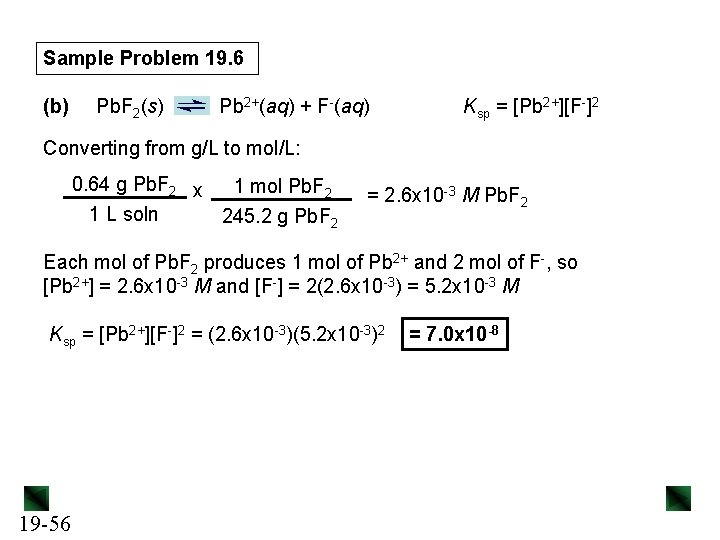Sample Problem 19. 6 (b) Pb. F 2(s) Pb 2+(aq) + F-(aq) Ksp = [Pb 2+][F-]2 Converting from g/L to mol/L: 0. 64 g Pb. F 2 x 1 mol Pb. F 2 1 L soln 245. 2 g Pb. F 2 = 2. 6 x 10 -3 M Pb. F 2 Each mol of Pb. F 2 produces 1 mol of Pb 2+ and 2 mol of F-, so [Pb 2+] = 2. 6 x 10 -3 M and [F-] = 2(2. 6 x 10 -3) = 5. 2 x 10 -3 M Ksp = [Pb 2+][F-]2 = (2. 6 x 10 -3)(5. 2 x 10 -3)2 19 -56 = 7. 0 x 10 -8Sample Problem 19. 7 Determining Solubility from Ksp PROBLEM: Calcium hydroxide (slaked lime) is a major component of mortar, plaster, and cement, and solutions of Ca(OH)2 are used in industry as a strong, inexpensive base. Calculate the molar solubility of Ca(OH)2 in water if the Ksp is 6. 5 x 10 -6. PLAN: We write the dissolution equation and the expression for Ksp. We know the value of Ksp, so we set up a reaction table that expresses [Ca 2+] and [OH-] in terms of S, the molar solubility. We then substitute these expressions into the Ksp expression and solve for S. SOLUTION: Ca(OH)2(s) 19 -57 Ca 2+(aq) + 2 OH-(aq) Ksp = [Ca 2+][OH-]2 = 6. 5 x 10 -6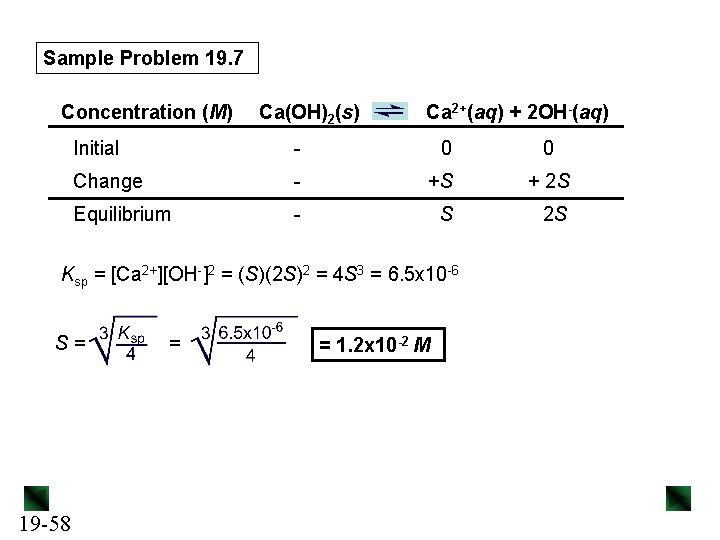Sample Problem 19. 7 Concentration (M) Ca(OH)2(s) Ca 2+(aq) + 2 OH-(aq) Initial - 0 Change - +S + 2 S Equilibrium - S 2 S Ksp = [Ca 2+][OH-]2 = (S)(2 S)2 = 4 S 3 = 6. 5 x 10 -6 S= 19 -58 = = 1. 2 x 10 -2 M 0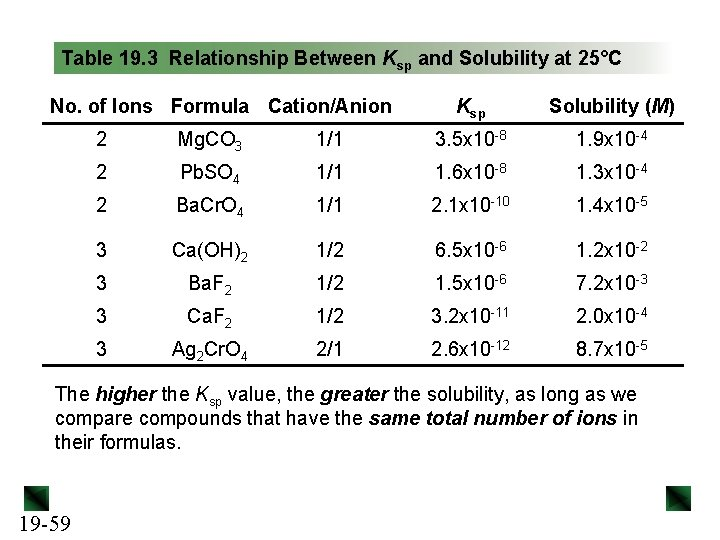Table 19. 3 Relationship Between Ksp and Solubility at 25°C No. of Ions Formula Cation/Anion Ksp Solubility (M) 2 Mg. CO 3 1/1 3. 5 x 10 -8 1. 9 x 10 -4 2 Pb. SO 4 1/1 1. 6 x 10 -8 1. 3 x 10 -4 2 Ba. Cr. O 4 1/1 2. 1 x 10 -10 1. 4 x 10 -5 3 Ca(OH)2 1/2 6. 5 x 10 -6 1. 2 x 10 -2 3 Ba. F 2 1/2 1. 5 x 10 -6 7. 2 x 10 -3 3 Ca. F 2 1/2 3. 2 x 10 -11 2. 0 x 10 -4 3 Ag 2 Cr. O 4 2/1 2. 6 x 10 -12 8. 7 x 10 -5 The higher the Ksp value, the greater the solubility, as long as we compare compounds that have the same total number of ions in their formulas. 19 -59Figure 19. 12 The effect of a common ion on solubility. Pb. Cr. O 4(s) Pb 2+(aq) + Cr. O 42 -(aq) If Na 2 Cr. O 4 solution is added to a saturated solution of Pb. Cr. O 4, it provides the common ion Cr. O 42 -, causing the equilibrium to shift to the left. Solubility decreases and solid Pb. Cr. O 4 precipitates. 19 -60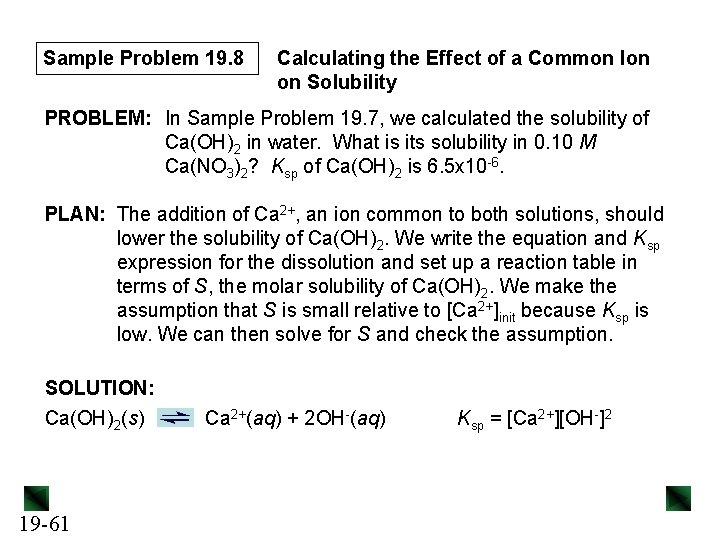Sample Problem 19. 8 Calculating the Effect of a Common Ion on Solubility PROBLEM: In Sample Problem 19. 7, we calculated the solubility of Ca(OH)2 in water. What is its solubility in 0. 10 M Ca(NO 3)2? Ksp of Ca(OH)2 is 6. 5 x 10 -6. PLAN: The addition of Ca 2+, an ion common to both solutions, should lower the solubility of Ca(OH)2. We write the equation and Ksp expression for the dissolution and set up a reaction table in terms of S, the molar solubility of Ca(OH)2. We make the assumption that S is small relative to [Ca 2+]init because Ksp is low. We can then solve for S and check the assumption. SOLUTION: Ca(OH)2(s) 19 -61 Ca 2+(aq) + 2 OH-(aq) Ksp = [Ca 2+][OH-]2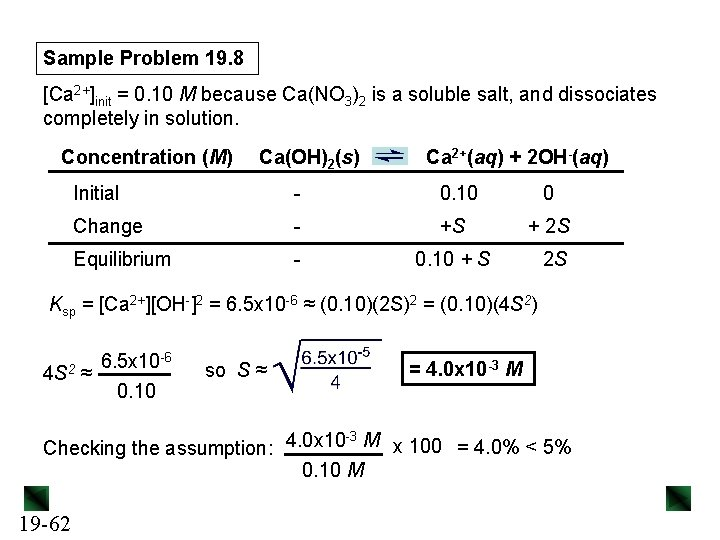Sample Problem 19. 8 [Ca 2+]init = 0. 10 M because Ca(NO 3)2 is a soluble salt, and dissociates completely in solution. Concentration (M) Ca(OH)2(s) Ca 2+(aq) + 2 OH-(aq) Initial - 0. 10 0 Change - +S + 2 S Equilibrium - 0. 10 + S 2 S Ksp = [Ca 2+][OH-]2 = 6. 5 x 10 -6 ≈ (0. 10)(2 S)2 = (0. 10)(4 S 2) 4 S 2 6. 5 x 10 -6 ≈ 0. 10 so S ≈ = 4. 0 x 10 -3 M -3 Checking the assumption: 4. 0 x 10 M x 100 = 4. 0% < 5% 0. 10 M 19 -62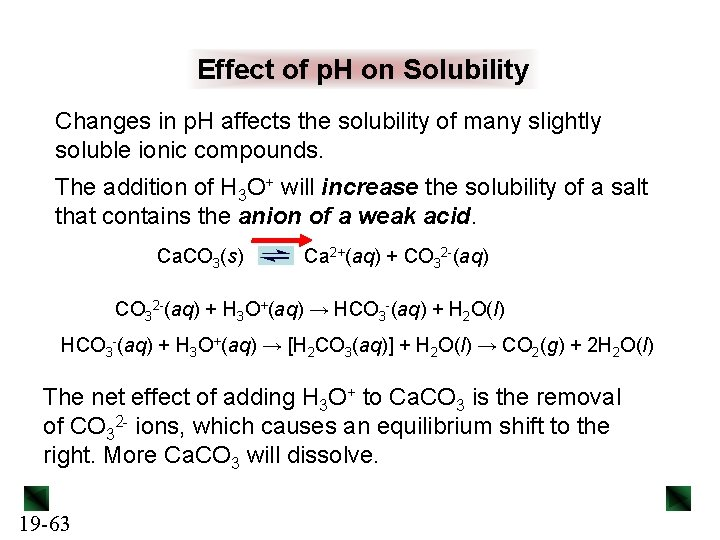Effect of p. H on Solubility Changes in p. H affects the solubility of many slightly soluble ionic compounds. The addition of H 3 O+ will increase the solubility of a salt that contains the anion of a weak acid. Ca. CO 3(s) Ca 2+(aq) + CO 32 -(aq) + H 3 O+(aq) → HCO 3 -(aq) + H 2 O(l) HCO 3 -(aq) + H 3 O+(aq) → [H 2 CO 3(aq)] + H 2 O(l) → CO 2(g) + 2 H 2 O(l) The net effect of adding H 3 O+ to Ca. CO 3 is the removal of CO 32 - ions, which causes an equilibrium shift to the right. More Ca. CO 3 will dissolve. 19 -63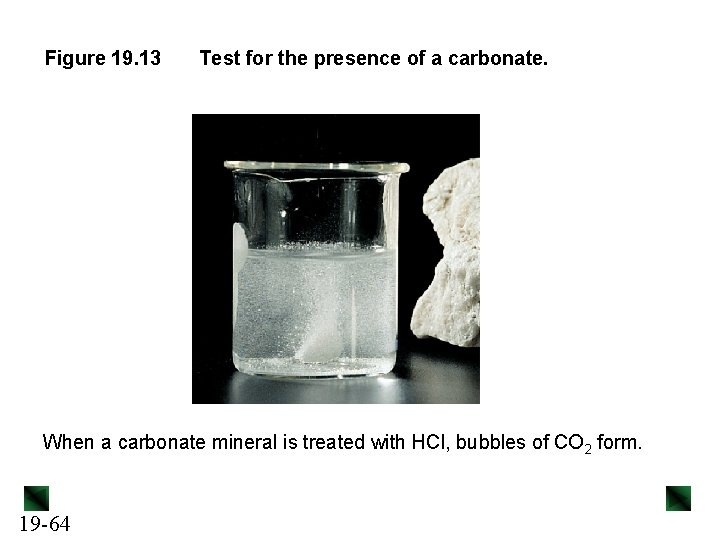Figure 19. 13 Test for the presence of a carbonate. When a carbonate mineral is treated with HCl, bubbles of CO 2 form. 19 -64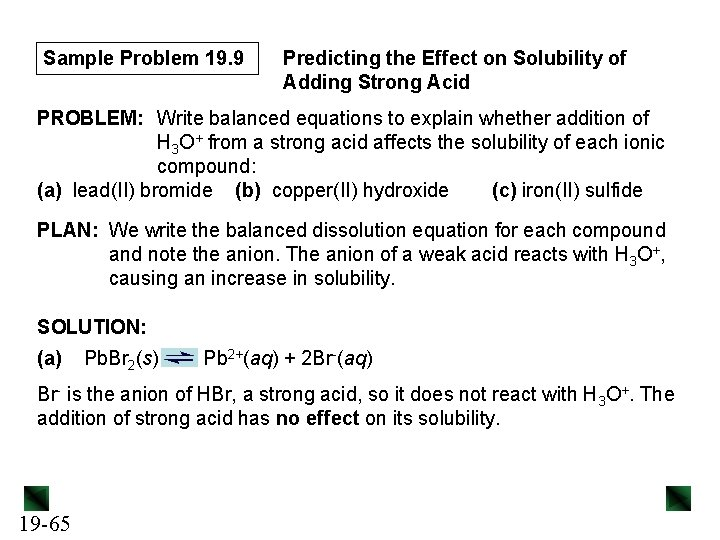Sample Problem 19. 9 Predicting the Effect on Solubility of Adding Strong Acid PROBLEM: Write balanced equations to explain whether addition of H 3 O+ from a strong acid affects the solubility of each ionic compound: (a) lead(II) bromide (b) copper(II) hydroxide (c) iron(II) sulfide PLAN: We write the balanced dissolution equation for each compound and note the anion. The anion of a weak acid reacts with H 3 O+, causing an increase in solubility. SOLUTION: (a) Pb. Br 2(s) Pb 2+(aq) + 2 Br-(aq) Br- is the anion of HBr, a strong acid, so it does not react with H 3 O+. The addition of strong acid has no effect on its solubility. 19 -65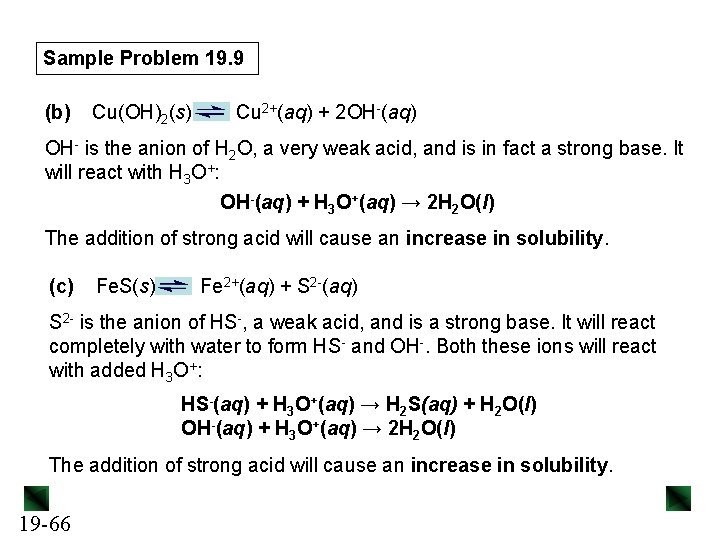Sample Problem 19. 9 (b) Cu(OH)2(s) Cu 2+(aq) + 2 OH-(aq) OH- is the anion of H 2 O, a very weak acid, and is in fact a strong base. It will react with H 3 O+: OH-(aq) + H 3 O+(aq) → 2 H 2 O(l) The addition of strong acid will cause an increase in solubility. (c) Fe. S(s) Fe 2+(aq) + S 2 -(aq) S 2 - is the anion of HS-, a weak acid, and is a strong base. It will react completely with water to form HS- and OH-. Both these ions will react with added H 3 O+: HS-(aq) + H 3 O+(aq) → H 2 S(aq) + H 2 O(l) OH-(aq) + H 3 O+(aq) → 2 H 2 O(l) The addition of strong acid will cause an increase in solubility. 19 -66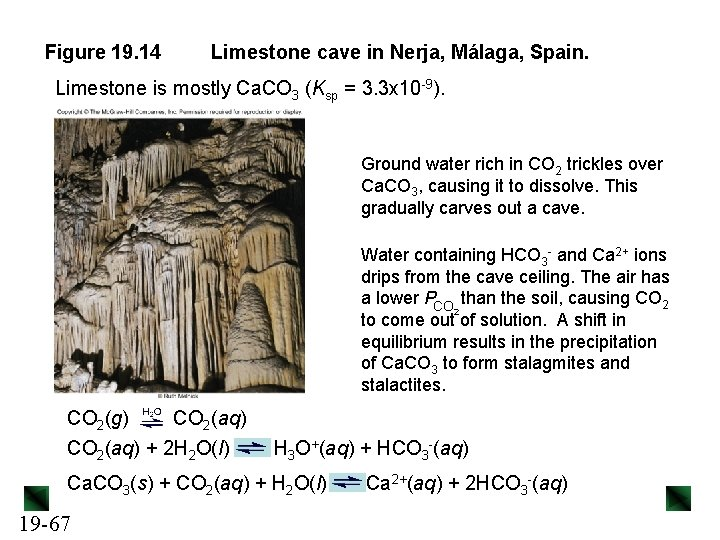Figure 19. 14 Limestone cave in Nerja, Málaga, Spain. Limestone is mostly Ca. CO 3 (Ksp = 3. 3 x 10 -9). Ground water rich in CO 2 trickles over Ca. CO 3, causing it to dissolve. This gradually carves out a cave. Water containing HCO 3 - and Ca 2+ ions drips from the cave ceiling. The air has a lower PCO than the soil, causing CO 2 2 to come out of solution. A shift in equilibrium results in the precipitation of Ca. CO 3 to form stalagmites and stalactites. CO 2(g) CO 2(aq) + 2 H 2 O(l) H 3 O+(aq) + HCO 3 -(aq) Ca. CO 3(s) + CO 2(aq) + H 2 O(l) 19 -67 Ca 2+(aq) + 2 HCO 3 -(aq)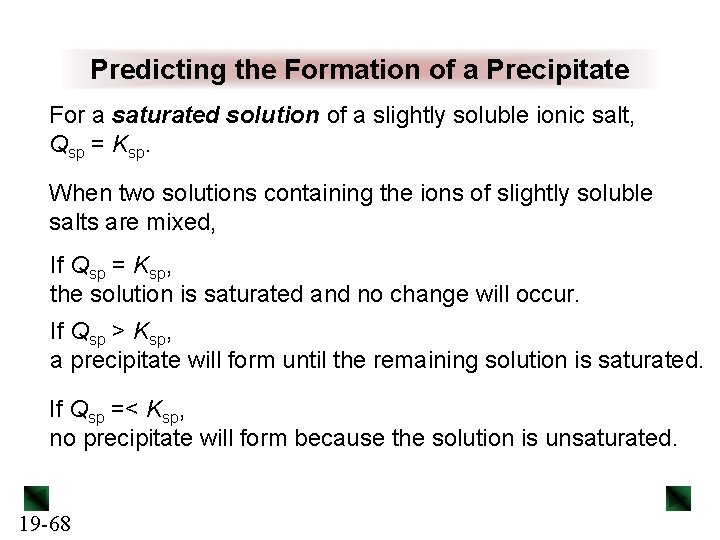Predicting the Formation of a Precipitate For a saturated solution of a slightly soluble ionic salt, Qsp = Ksp. When two solutions containing the ions of slightly soluble salts are mixed, If Qsp = Ksp, the solution is saturated and no change will occur. If Qsp > Ksp, a precipitate will form until the remaining solution is saturated. If Qsp =< Ksp, no precipitate will form because the solution is unsaturated. 19 -68Sample Problem 19. 10 Predicting Whether a Precipitate Will Form PROBLEM: A common laboratory method for preparing a precipitate is to mix solutions containing the component ions. Does a precipitate form when 0. 100 L of 0. 30 M Ca(NO 3)2 is mixed with 0. 200 L of 0. 060 M Na. F? PLAN: First we need to decide which slightly soluble salt could form, look up its Ksp value in Appendix C, and write the dissolution equation and Ksp expression. We find the initial ion concentrations from the given volumes and molarities of the two solutions, calculate the value for Qsp and compare it to Ksp. SOLUTION: The ions present are Ca 2+, NO 3 -, Na+, and F-. All Na+ and NO 3 - salts are soluble, so the only possible precipitate is Ca. F 2 (Ksp = 3. 2 x 10 -11). Ca. F 2(s) 19 -69 Ca 2+(aq) + 2 F-(aq) Ksp = [Ca 2+][F-]2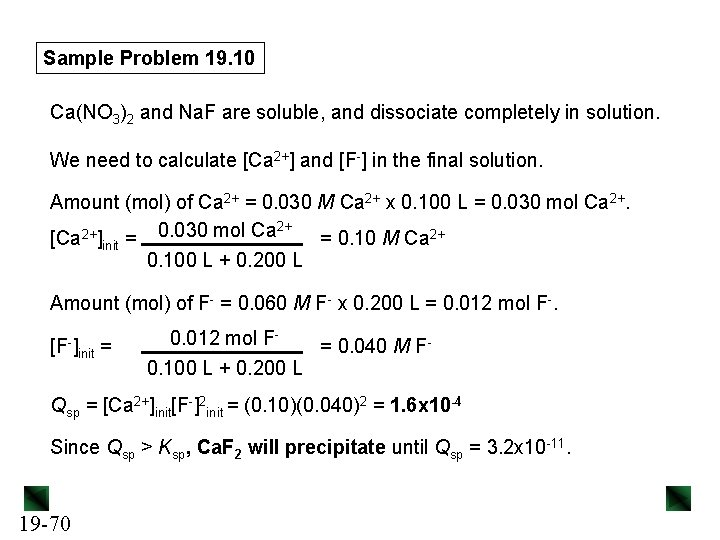Sample Problem 19. 10 Ca(NO 3)2 and Na. F are soluble, and dissociate completely in solution. We need to calculate [Ca 2+] and [F-] in the final solution. Amount (mol) of Ca 2+ = 0. 030 M Ca 2+ x 0. 100 L = 0. 030 mol Ca 2+. 2+ 0. 030 mol Ca 2+ [Ca ]init = = 0. 10 M Ca 2+ 0. 100 L + 0. 200 L Amount (mol) of F- = 0. 060 M F- x 0. 200 L = 0. 012 mol F-. [F-] init = 0. 012 mol F= 0. 040 M F 0. 100 L + 0. 200 L Qsp = [Ca 2+]init[F-]2 init = (0. 10)(0. 040)2 = 1. 6 x 10 -4 Since Qsp > Ksp, Ca. F 2 will precipitate until Qsp = 3. 2 x 10 -11. 19 -70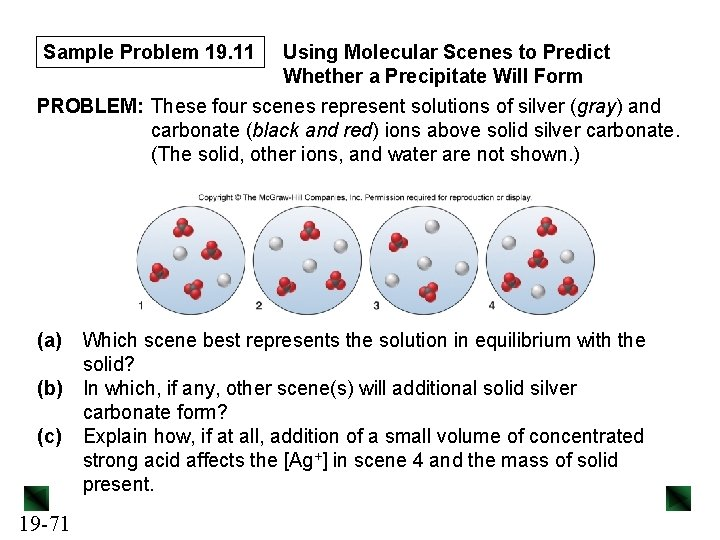Sample Problem 19. 11 Using Molecular Scenes to Predict Whether a Precipitate Will Form PROBLEM: These four scenes represent solutions of silver (gray) and carbonate (black and red) ions above solid silver carbonate. (The solid, other ions, and water are not shown. ) (a) Which scene best represents the solution in equilibrium with the solid? (b) In which, if any, other scene(s) will additional solid silver carbonate form? (c) Explain how, if at all, addition of a small volume of concentrated strong acid affects the [Ag+] in scene 4 and the mass of solid present. 19 -71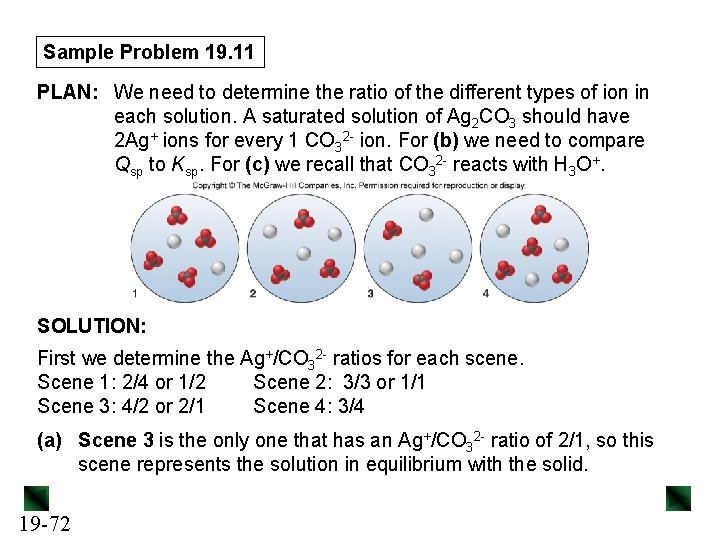Sample Problem 19. 11 PLAN: We need to determine the ratio of the different types of ion in each solution. A saturated solution of Ag 2 CO 3 should have 2 Ag+ ions for every 1 CO 32 - ion. For (b) we need to compare Qsp to Ksp. For (c) we recall that CO 32 - reacts with H 3 O+. SOLUTION: First we determine the Ag+/CO 32 - ratios for each scene. Scene 1: 2/4 or 1/2 Scene 2: 3/3 or 1/1 Scene 3: 4/2 or 2/1 Scene 4: 3/4 (a) Scene 3 is the only one that has an Ag+/CO 32 - ratio of 2/1, so this scene represents the solution in equilibrium with the solid. 19 -72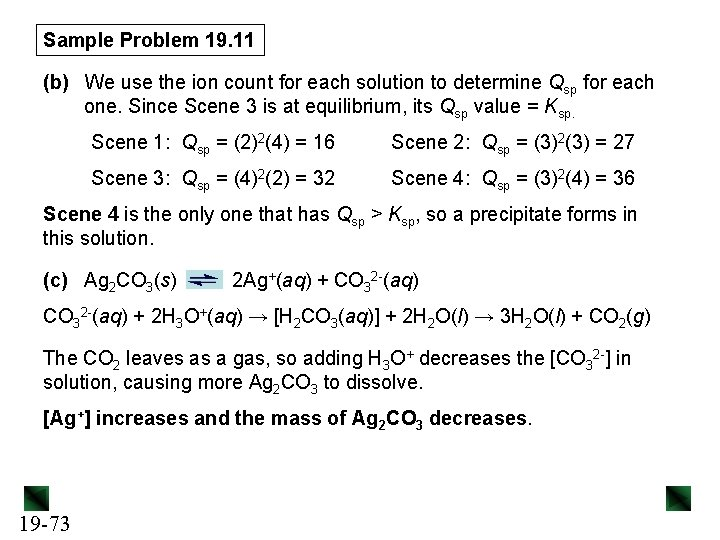Sample Problem 19. 11 (b) We use the ion count for each solution to determine Qsp for each one. Since Scene 3 is at equilibrium, its Qsp value = Ksp. Scene 1: Qsp = (2)2(4) = 16 Scene 2: Qsp = (3)2(3) = 27 Scene 3: Qsp = (4)2(2) = 32 Scene 4: Qsp = (3)2(4) = 36 Scene 4 is the only one that has Qsp > Ksp, so a precipitate forms in this solution. (c) Ag 2 CO 3(s) 2 Ag+(aq) + CO 32 -(aq) + 2 H 3 O+(aq) → [H 2 CO 3(aq)] + 2 H 2 O(l) → 3 H 2 O(l) + CO 2(g) The CO 2 leaves as a gas, so adding H 3 O+ decreases the [CO 32 -] in solution, causing more Ag 2 CO 3 to dissolve. [Ag+] increases and the mass of Ag 2 CO 3 decreases. 19 -73Selective Precipitation Selective precipitation is used to separate a solution containing a mixture of ions. A precipitating ion is added to the solution until the Qsp of the more soluble compound is almost equal to its Ksp. The less soluble compound will precipitate in as large a quantity as possible, leaving behind the ion of the more soluble compound. 19 -74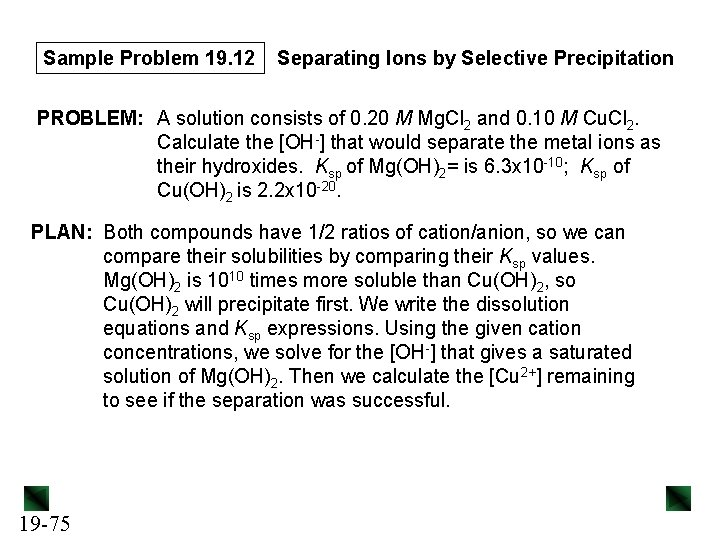Sample Problem 19. 12 Separating Ions by Selective Precipitation PROBLEM: A solution consists of 0. 20 M Mg. Cl 2 and 0. 10 M Cu. Cl 2. Calculate the [OH-] that would separate the metal ions as their hydroxides. Ksp of Mg(OH)2= is 6. 3 x 10 -10; Ksp of Cu(OH)2 is 2. 2 x 10 -20. PLAN: Both compounds have 1/2 ratios of cation/anion, so we can compare their solubilities by comparing their Ksp values. Mg(OH)2 is 1010 times more soluble than Cu(OH)2, so Cu(OH)2 will precipitate first. We write the dissolution equations and Ksp expressions. Using the given cation concentrations, we solve for the [OH-] that gives a saturated solution of Mg(OH)2. Then we calculate the [Cu 2+] remaining to see if the separation was successful. 19 -75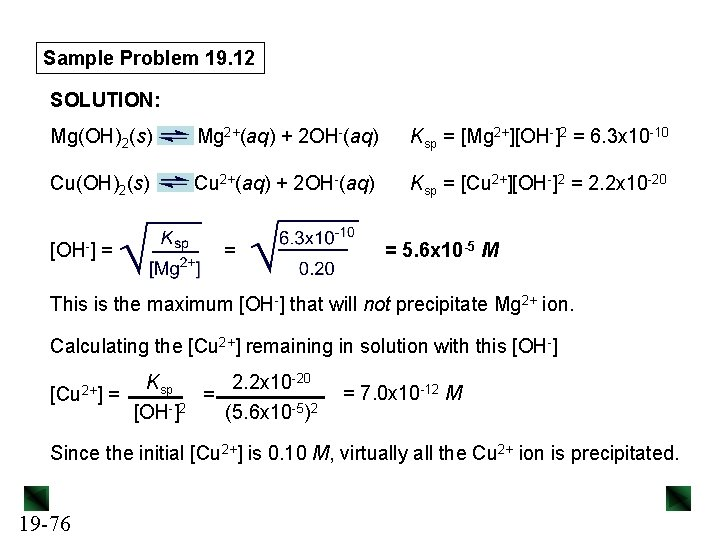Sample Problem 19. 12 SOLUTION: Mg(OH)2(s) Mg 2+(aq) + 2 OH-(aq) Ksp = [Mg 2+][OH-]2 = 6. 3 x 10 -10 Cu(OH)2(s) Cu 2+(aq) + 2 OH-(aq) Ksp = [Cu 2+][OH-]2 = 2. 2 x 10 -20 [OH-] = = = 5. 6 x 10 -5 M This is the maximum [OH-] that will not precipitate Mg 2+ ion. Calculating the [Cu 2+] remaining in solution with this [OH-] [Cu 2+] Ksp 2. 2 x 10 -20 = = 2 [OH ] (5. 6 x 10 -5)2 = 7. 0 x 10 -12 M Since the initial [Cu 2+] is 0. 10 M, virtually all the Cu 2+ ion is precipitated. 19 -76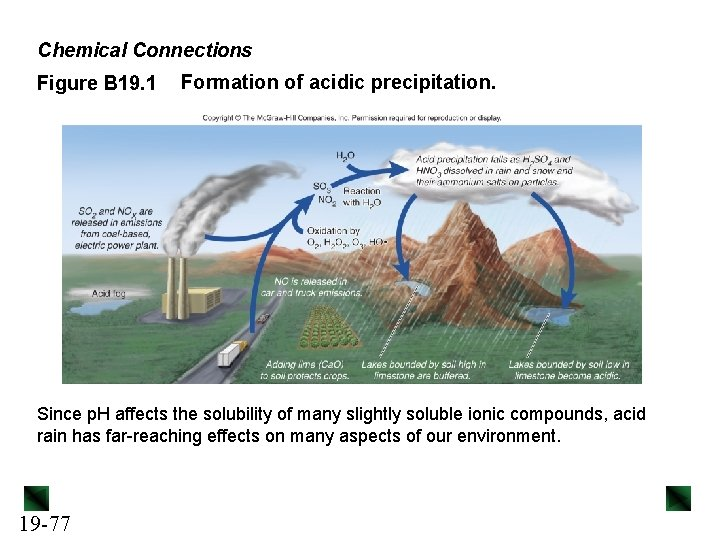Chemical Connections Figure B 19. 1 Formation of acidic precipitation. Since p. H affects the solubility of many slightly soluble ionic compounds, acid rain has far-reaching effects on many aspects of our environment. 19 -77Figure 19. 15 Cr(NH 3)63+, a typical complex ion. A complex ion consists of a central metal ion covalently bonded to two or more anions or molecules, called ligands. 19 -78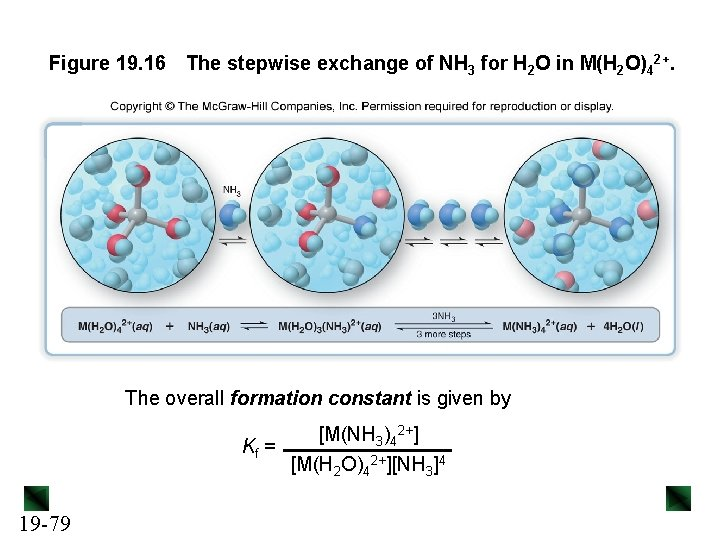Figure 19. 16 The stepwise exchange of NH 3 for H 2 O in M(H 2 O)42+. The overall formation constant is given by [M(NH 3)42+] Kf = [M(H 2 O)42+][NH 3]4 19 -79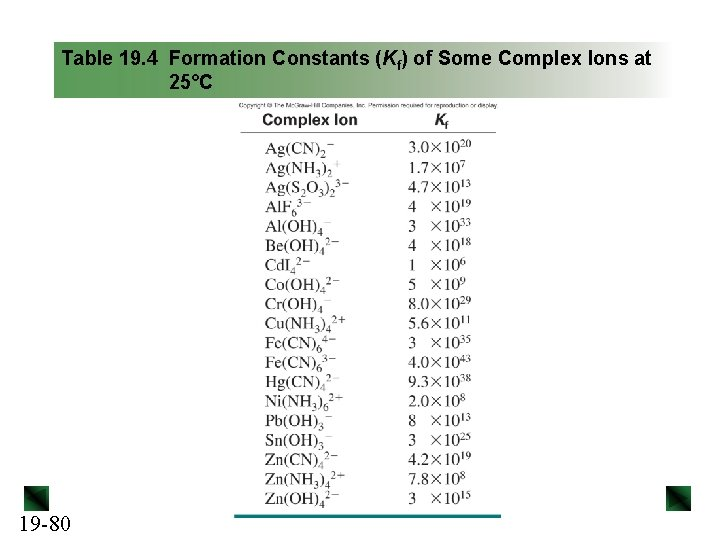Table 19. 4 Formation Constants (Kf) of Some Complex Ions at 25°C 19 -80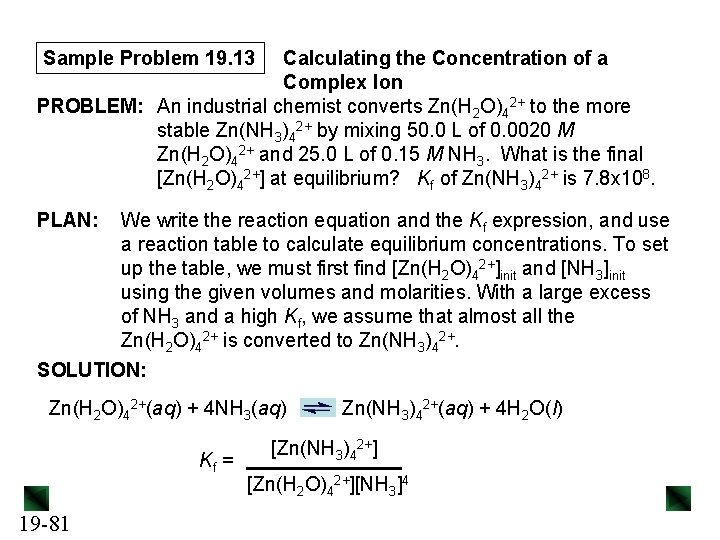Sample Problem 19. 13 Calculating the Concentration of a Complex Ion PROBLEM: An industrial chemist converts Zn(H 2 O)42+ to the more stable Zn(NH 3)42+ by mixing 50. 0 L of 0. 0020 M Zn(H 2 O)42+ and 25. 0 L of 0. 15 M NH 3. What is the final [Zn(H 2 O)42+] at equilibrium? Kf of Zn(NH 3)42+ is 7. 8 x 108. PLAN: We write the reaction equation and the Kf expression, and use a reaction table to calculate equilibrium concentrations. To set up the table, we must first find [Zn(H 2 O)42+]init and [NH 3]init using the given volumes and molarities. With a large excess of NH 3 and a high Kf, we assume that almost all the Zn(H 2 O)42+ is converted to Zn(NH 3)42+. SOLUTION: Zn(H 2 O)42+(aq) + 4 NH 3(aq) Kf = 19 -81 Zn(NH 3)42+(aq) + 4 H 2 O(l) [Zn(NH 3)42+] [Zn(H 2 O)42+][NH 3]4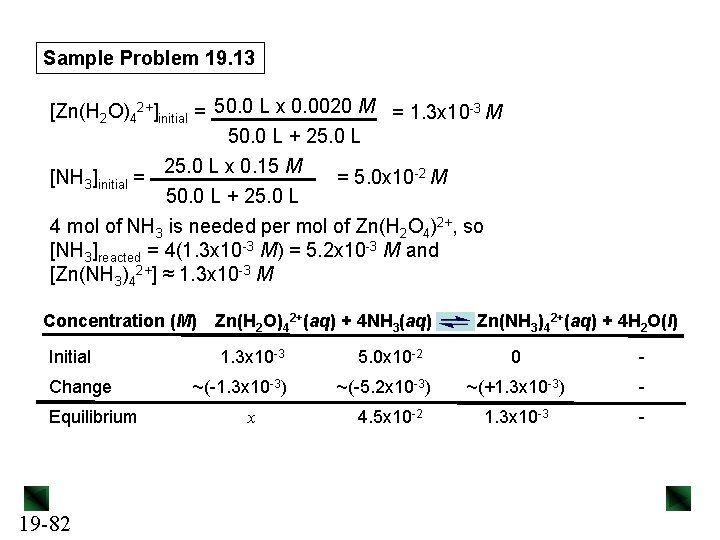Sample Problem 19. 13 [Zn(H 2 O)42+]initial = 50. 0 L x 0. 0020 M = 1. 3 x 10 -3 M 50. 0 L + 25. 0 L x 0. 15 M = 5. 0 x 10 -2 M 50. 0 L + 25. 0 L 4 mol of NH 3 is needed per mol of Zn(H 2 O 4)2+, so [NH 3]reacted = 4(1. 3 x 10 -3 M) = 5. 2 x 10 -3 M and [Zn(NH 3)42+] ≈ 1. 3 x 10 -3 M [NH 3]initial = Concentration (M) Initial Change Equilibrium 19 -82 Zn(H 2 O)42+(aq) + 4 NH 3(aq) 1. 3 x 10 -3 ~(-1. 3 x 10 -3) x 5. 0 x 10 -2 ~(-5. 2 x 10 -3) 4. 5 x 10 -2 Zn(NH 3)42+(aq) + 4 H 2 O(l) 0 - ~(+1. 3 x 10 -3) - 1. 3 x 10 -3 -Sample Problem 19. 13 Kf = [Zn(NH 3)42+] [Zn(H 2 O)42+][NH 3]4 = 7. 8 x 108 x = [Zn(H 2 O)42+ = 4. 1 x 10 -7 M 19 -83 = (1. 3 x 10 -3) x(4. 5 x 10 -2)4Sample Problem 19. 14 Calculating the Effect of Complex-Ion Formation on Solubility PROBLEM: In black-and-white film developing, excess Ag. Br is removed from the film negative by “hypo”, an aqueous solution of sodium thiosulfate (Na 2 S 2 O 3), which forms the complex ion Ag(S 2 O 3)23 -. Calculate the solubility of Ag. Br in (a) H 2 O; (b) 1. 0 M hypo. Kf of Ag(S 2 O 3)23 - is 4. 7 x 1013 and Ksp Ag. Br is 5. 0 x 10 -13. PLAN: After writing the equation and the Ksp expression, we use the given Ksp value to solve for S, the molar solubility of Ag. Br. For (b) we note that Ag. Br forms a complex ion with S 2 O 32 -, which shifts the equilibrium and dissolves more Ag. Br. We write an overall equation for the process and set up a reaction table to solve for S. 19 -84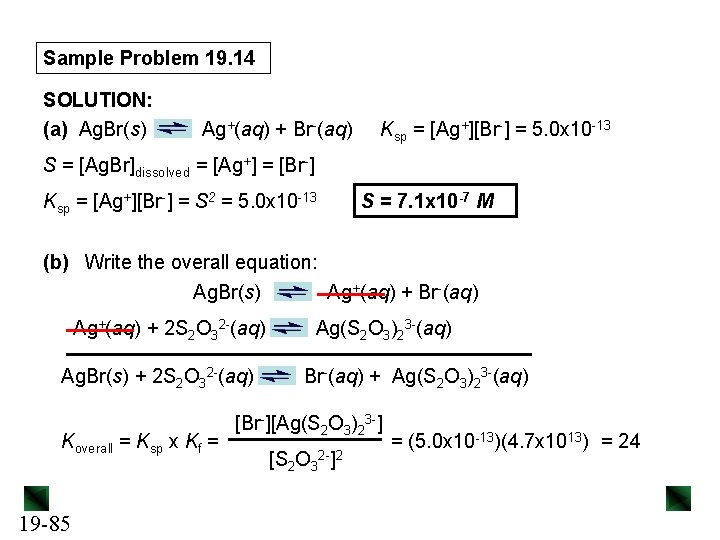Sample Problem 19. 14 SOLUTION: (a) Ag. Br(s) Ag+(aq) + Br-(aq) Ksp = [Ag+][Br-] = 5. 0 x 10 -13 S = [Ag. Br]dissolved = [Ag+] = [Br-] Ksp = [Ag+][Br-] = S 2 = 5. 0 x 10 -13 S = 7. 1 x 10 -7 M (b) Write the overall equation: Ag. Br(s) Ag+(aq) + Br-(aq) Ag+(aq) + 2 S 2 O 32 -(aq) Ag. Br(s) + 2 S 2 O 32 -(aq) Koverall = Ksp x Kf = 19 -85 Ag(S 2 O 3)23 -(aq) Br-(aq) + Ag(S 2 O 3)23 -(aq) [Br-][Ag(S 2 O 3)23 -] [S 2 O 32 -]2 = (5. 0 x 10 -13)(4. 7 x 1013) = 24Sample Problem 19. 14 Concentration (M) Ag. Br(s) + 2 S 2 O 32 -(aq) Initial Change Equilibrium Koverall = S 2 (1. 0 - 2 S)2 1. 0 -2 S 1. 0 - 2 S = 24 so S = 4. 9 M – 0. 9 S and 10. 9 S = 4. 9 M S = [Ag(S 2 O 3)23 -] = 0. 45 M 19 -86 Br-(aq) + Ag(S 2 O 3)23 -(aq) 0 +S S S 1. 0 - 2 S = 0 +S S = 4. 9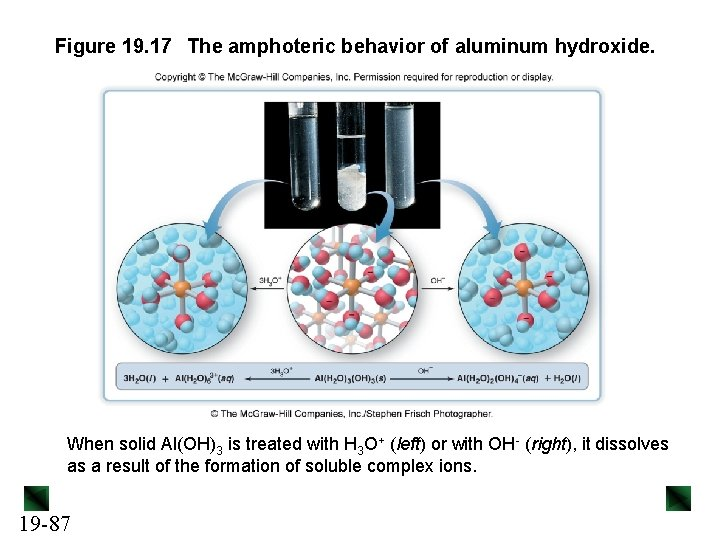Figure 19. 17 The amphoteric behavior of aluminum hydroxide. When solid Al(OH)3 is treated with H 3 O+ (left) or with OH- (right), it dissolves as a result of the formation of soluble complex ions. 19 -87